# 数据结构与算法： 可计算性问题

## (In)Tractability Problem

Posted by R1NG on May 1, 2022 Viewed Times

# 可计算性问题

## P, NP 和其他非确定性问题 (Intractable) 问题定义

### 直观定义

1. Class P: 任何可在 多项式时间内确定性算法 得出结论的问题都属于 P 类问题.
2. Class NP: 任何 需要使用非确定性算法 才能在多项式时间内求解, 可在 多项式时间内检验解答是否正确 的问题属于 NP 问题.
3. Class NP-Hard: 难度至少和所有的 NP 问题一样难 的问题属于 NP-Hard 问题.
4. Class NP-Complete: 既属于 NP 类, 又属于 NP-Hard 的问题为 NP-Hard 问题.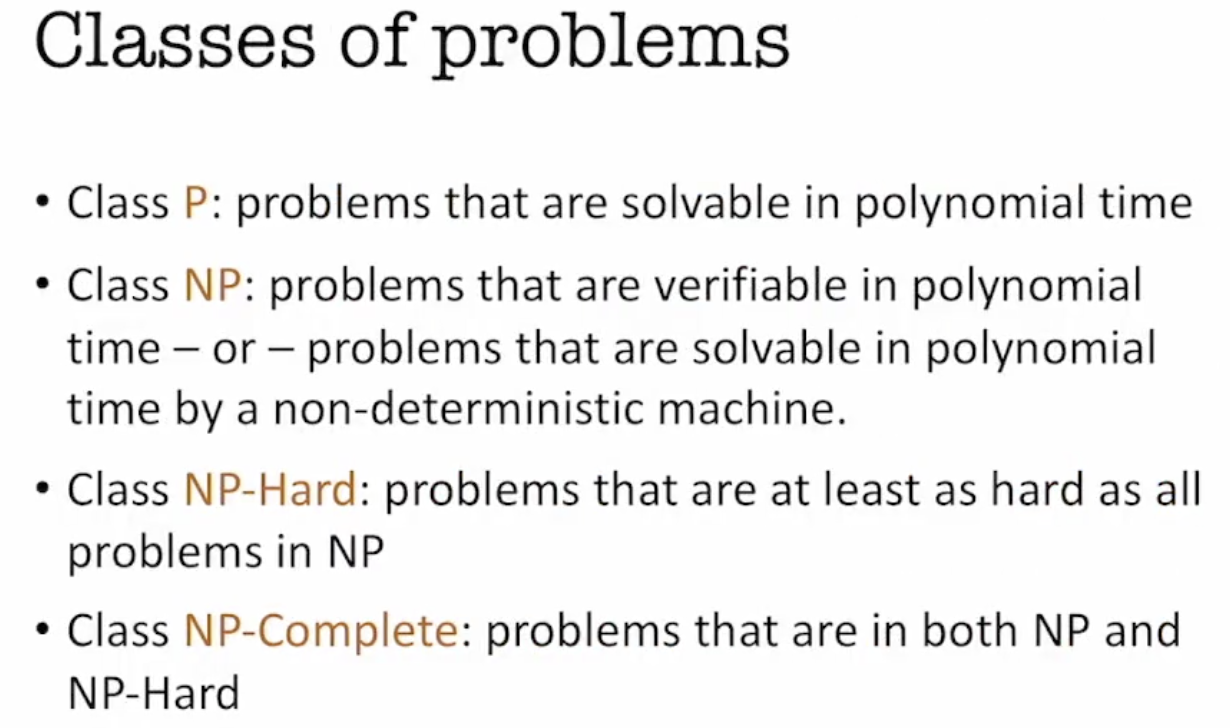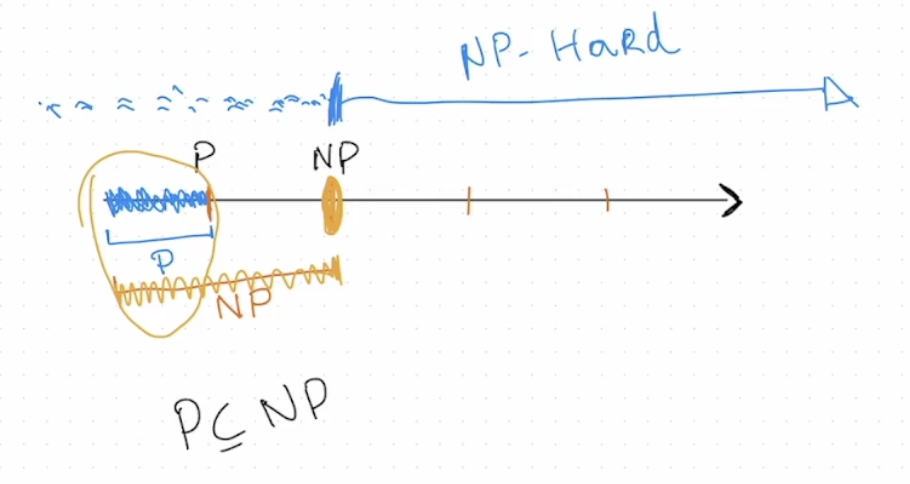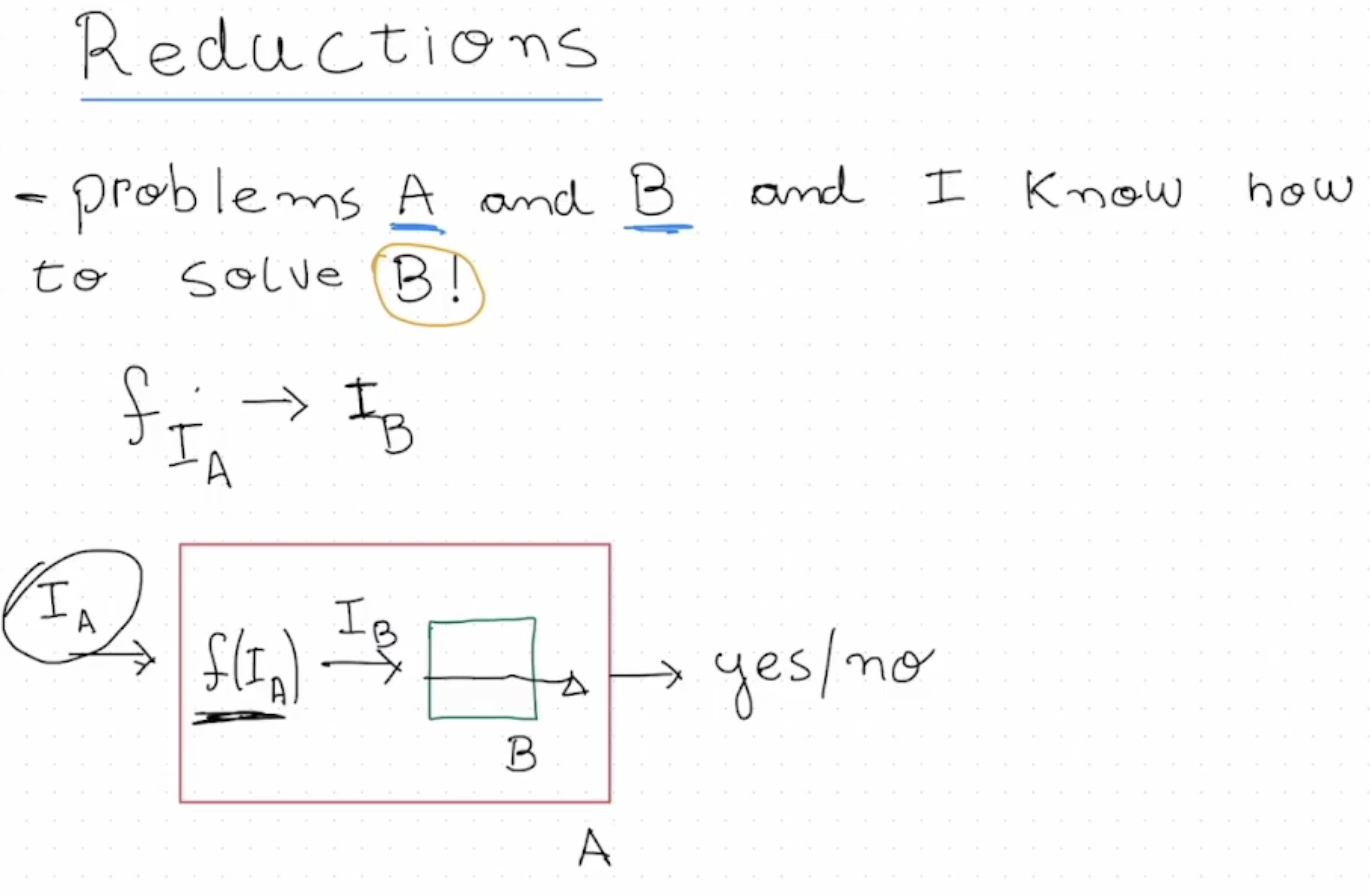### Class P 问题的形式化定义和对问题的编码

1. 一般来说优化问题都可以 等价地简化为 某个决策问题.
2. 解决决策问题一般不会比解决优化问题更难.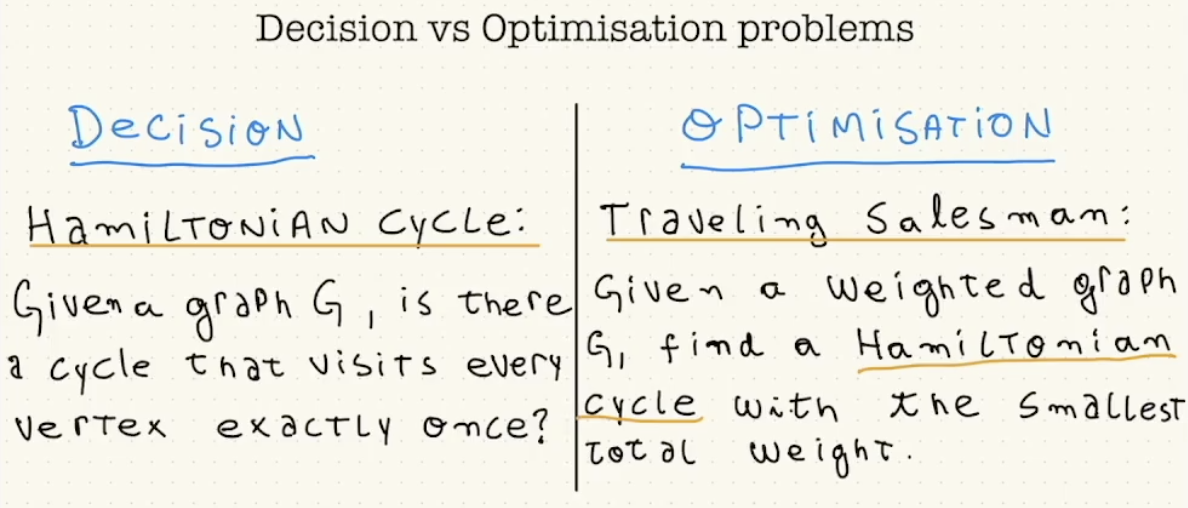#### 问题的统一编码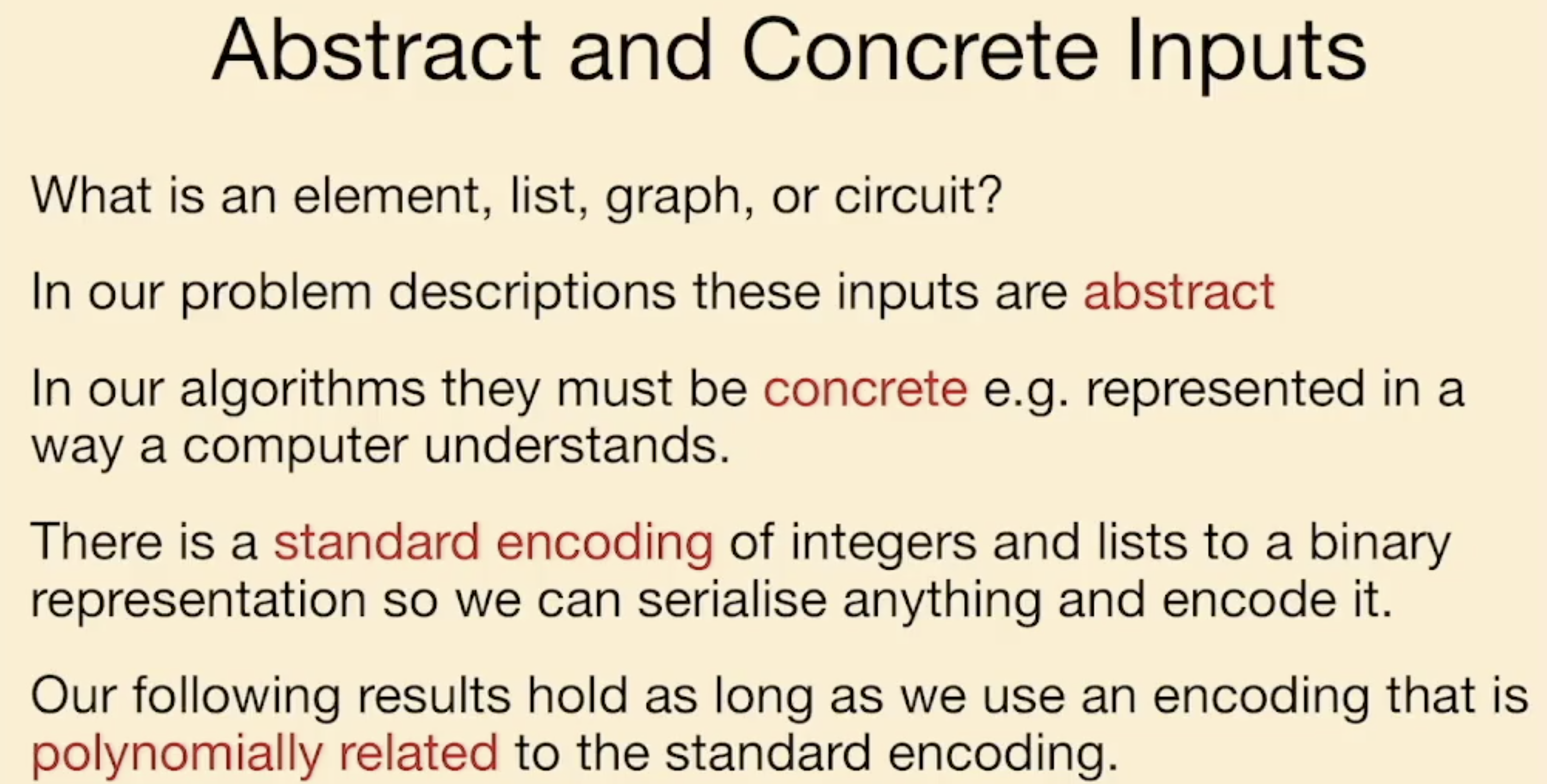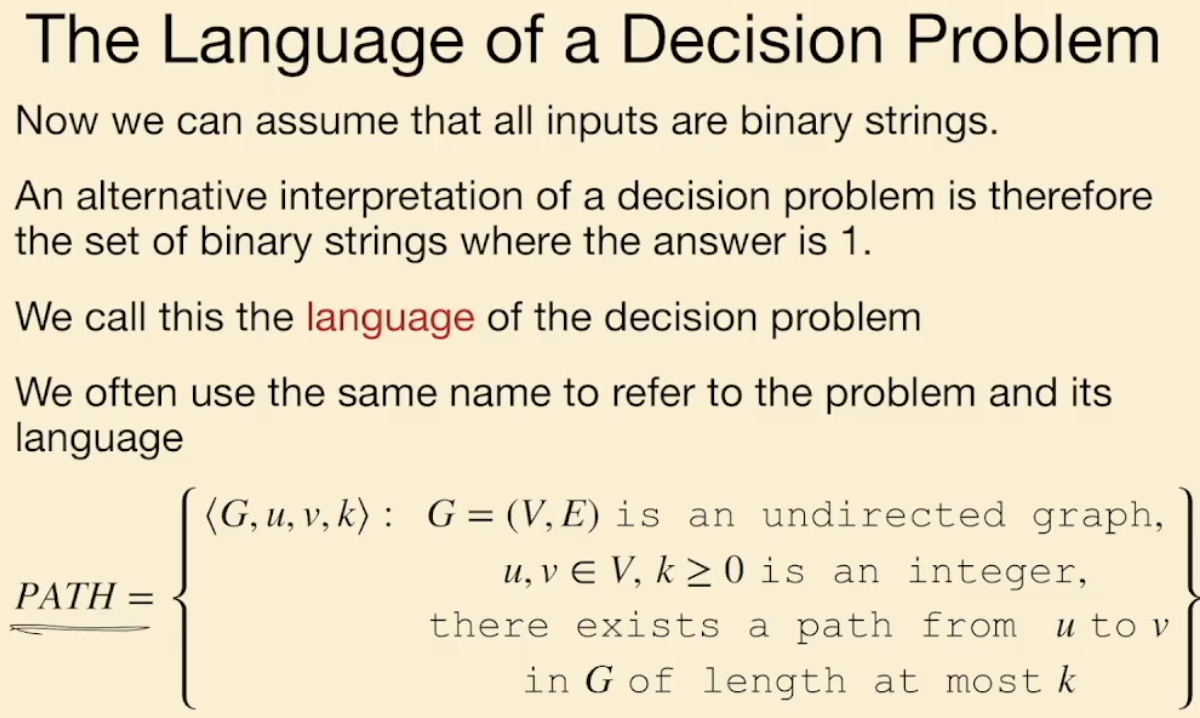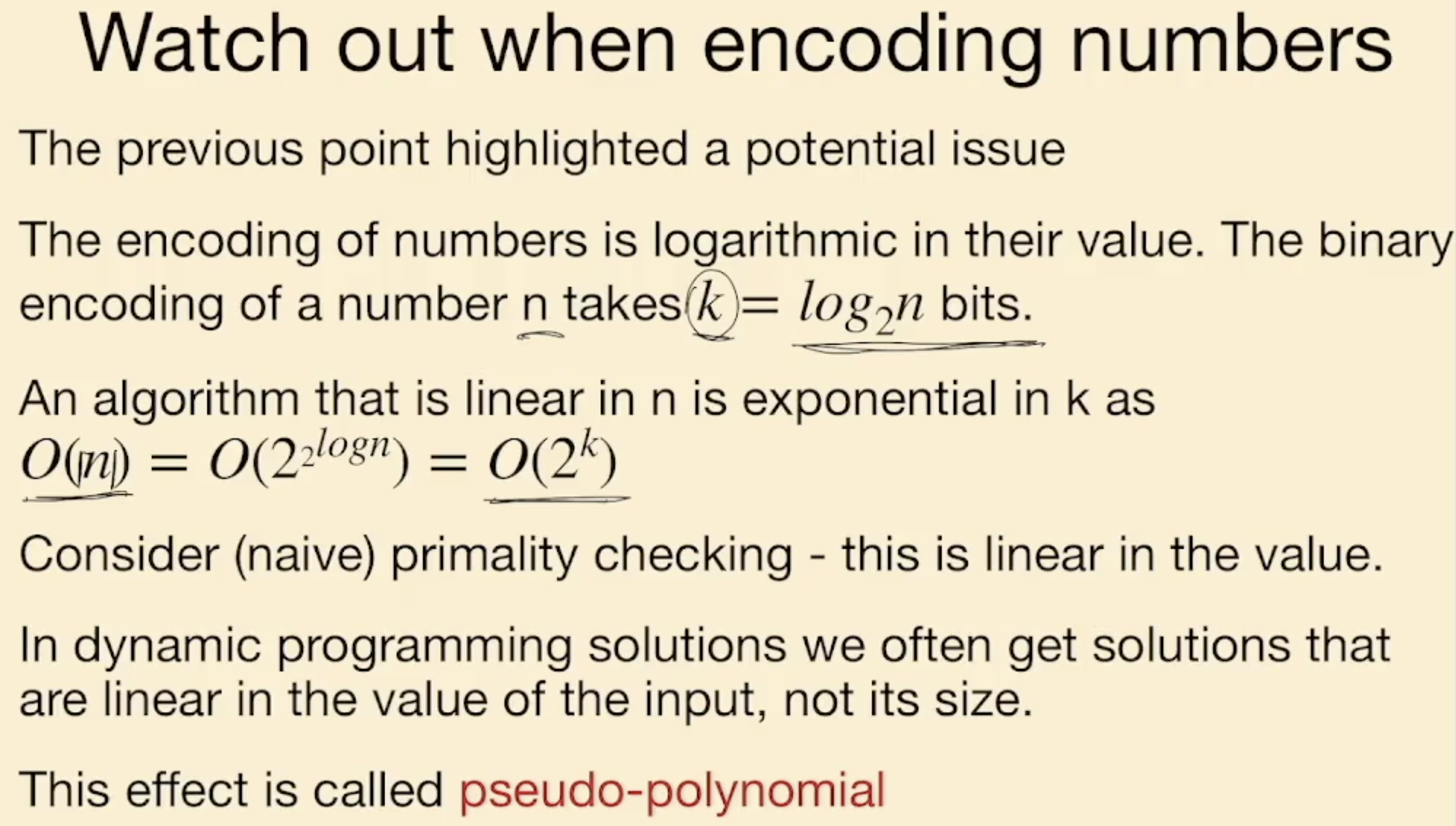#### Class P 问题的形式化定义

1. 记算法 $A$ 接纳 某个二进制串 $x$, 当且仅当满足 $A(x) = 1$.
2. 然后, 对任何 被问题的语言 $L$ 描述的二进制串 $x$, 若所有这样的 $x$ 都被 $A$ 接纳, 也就是

$A(x)=1 ~~ \text{for} ~ \forall x \in L$

$A(x)=0 ~~ \text{for} ~ \forall x \notin L$

则称 问题的语言 $L$ 被算法 $A$ 所确定.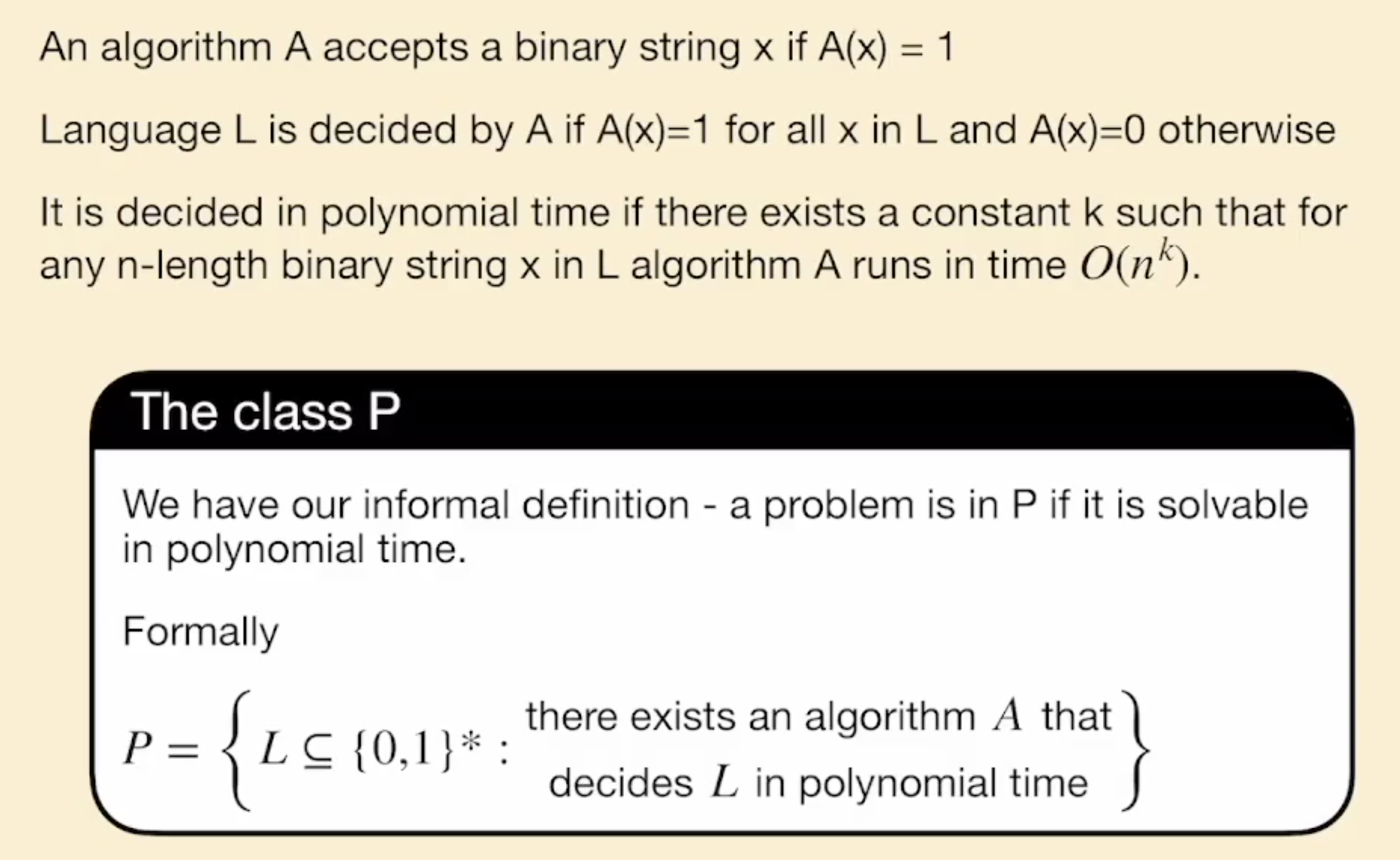### Class NP 问题的形式化定义与 Certificate 的定义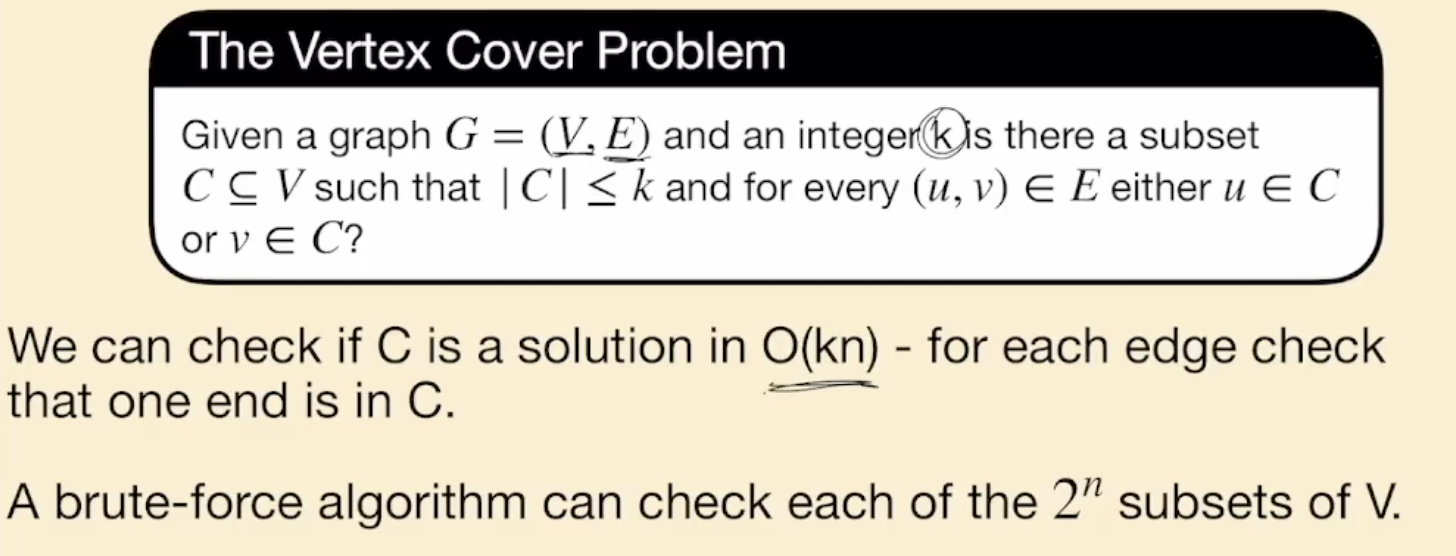k-SAT 问题关注的是 对每个子句中最多有 $k$ 个文字 的合取范式 (CNF) 的可满足性问题. (回顾 COMP21111)

1. SAT3-SAT 问题可互相转换, 并且它们都是 NP 问题, 而且其实它们也都是 NP-Complete 的.
2. 2-SAT 可在多项式时间内被求解.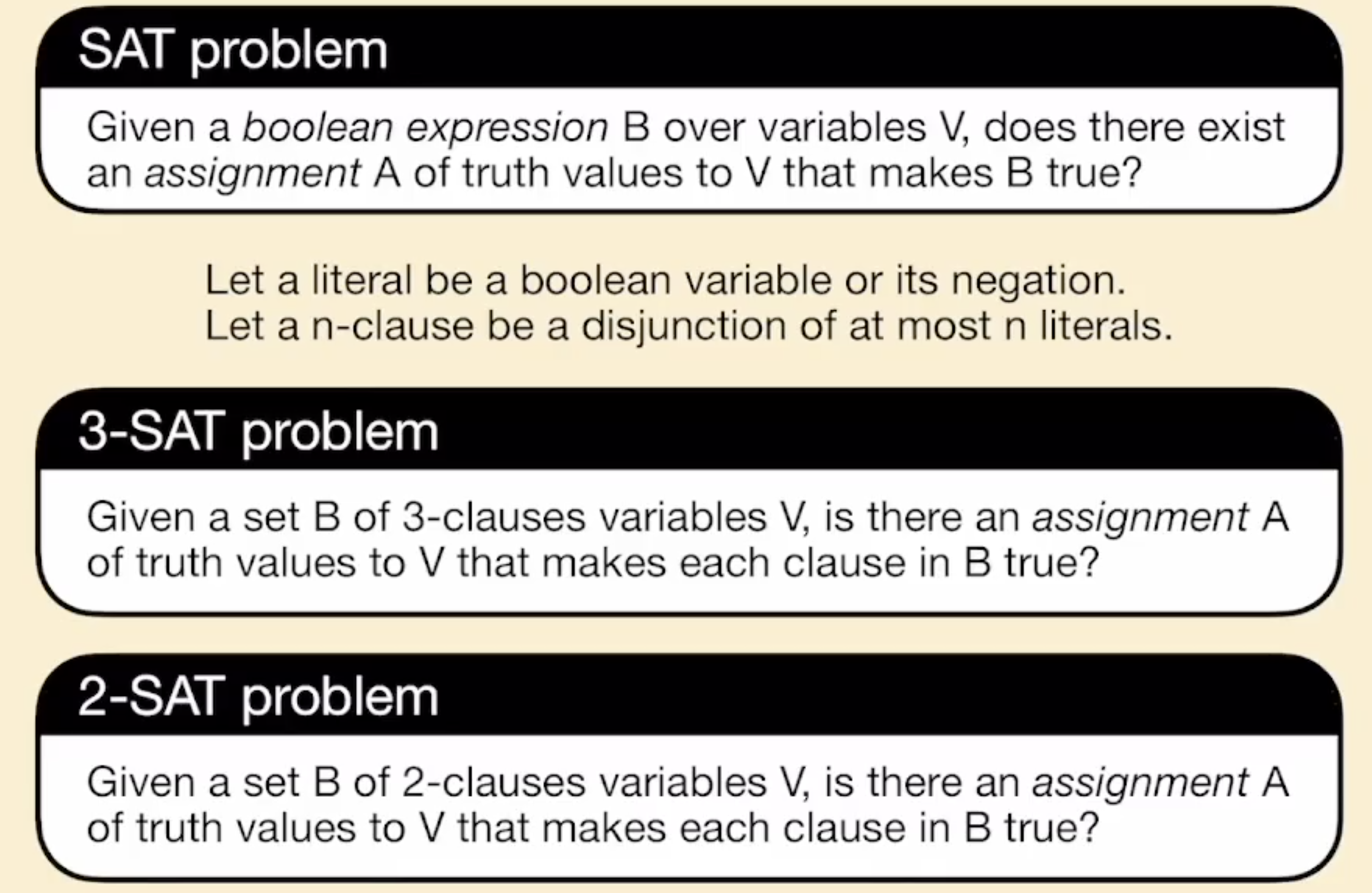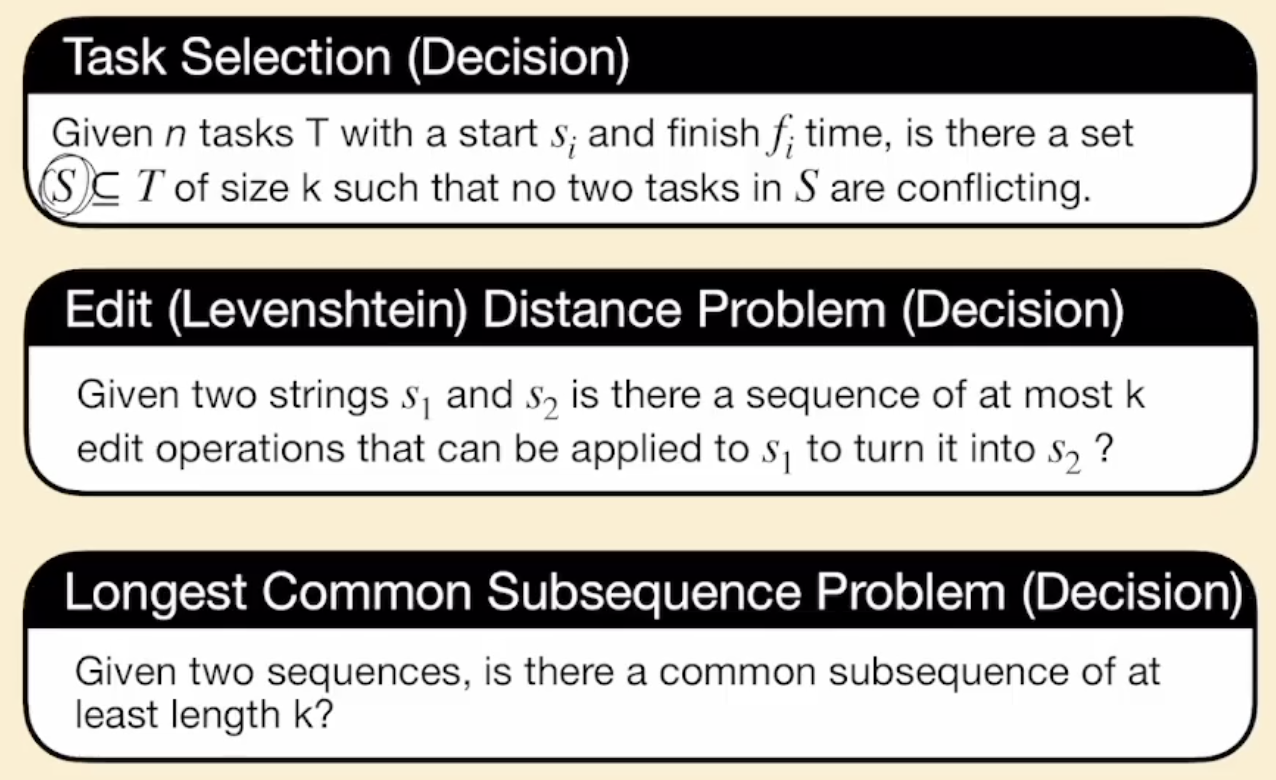1. 满足条件的集合 $S$.
2. 一系列长不超过 $k$ 的操作序列, 可被证明确实满足条件.
3. 现成的, 不超过 $k$ 的一个序列, 可被证明确实是两个字符串的公共序列.

Class NP 就是所有满足上述条件的问题语言组成的集合.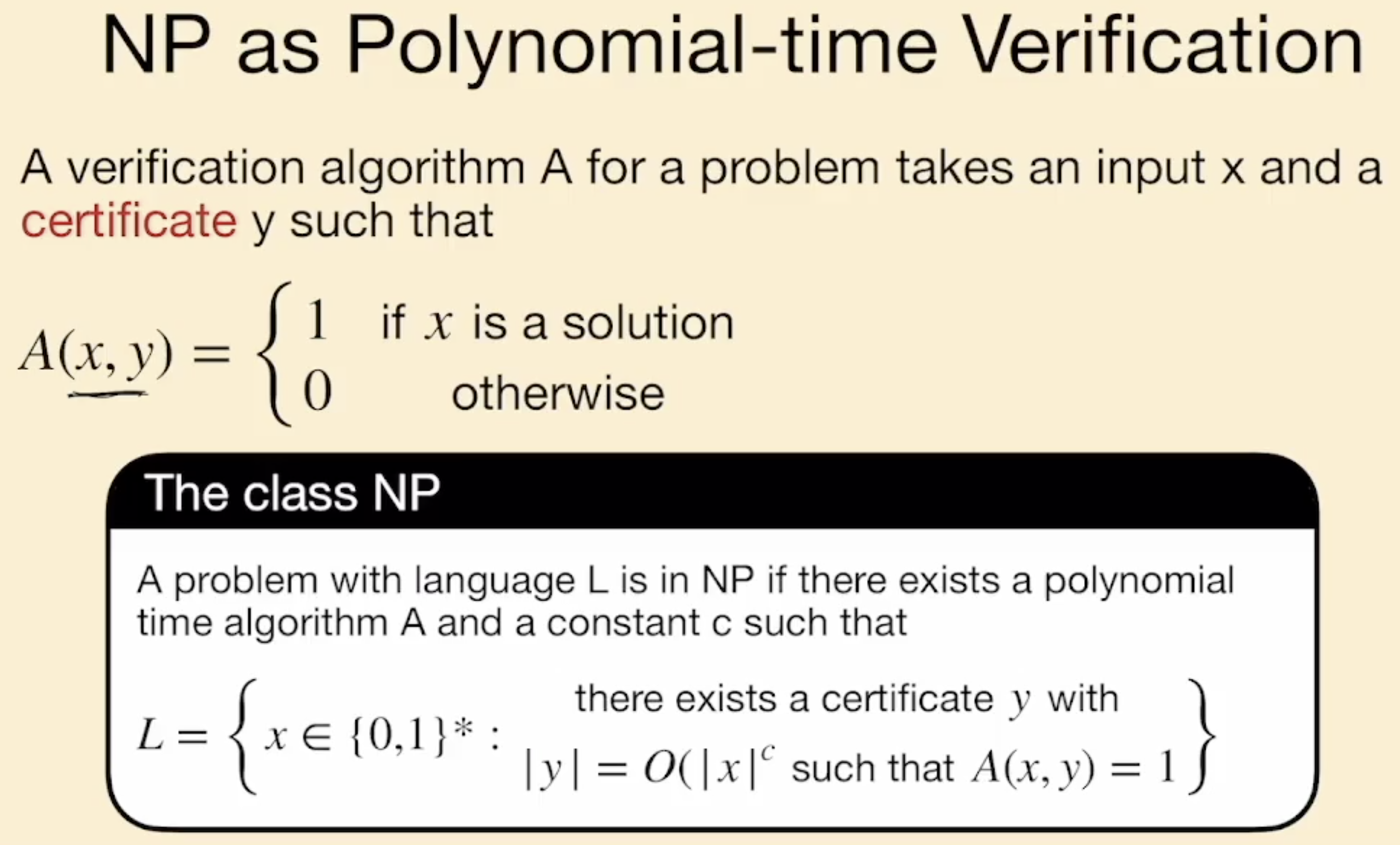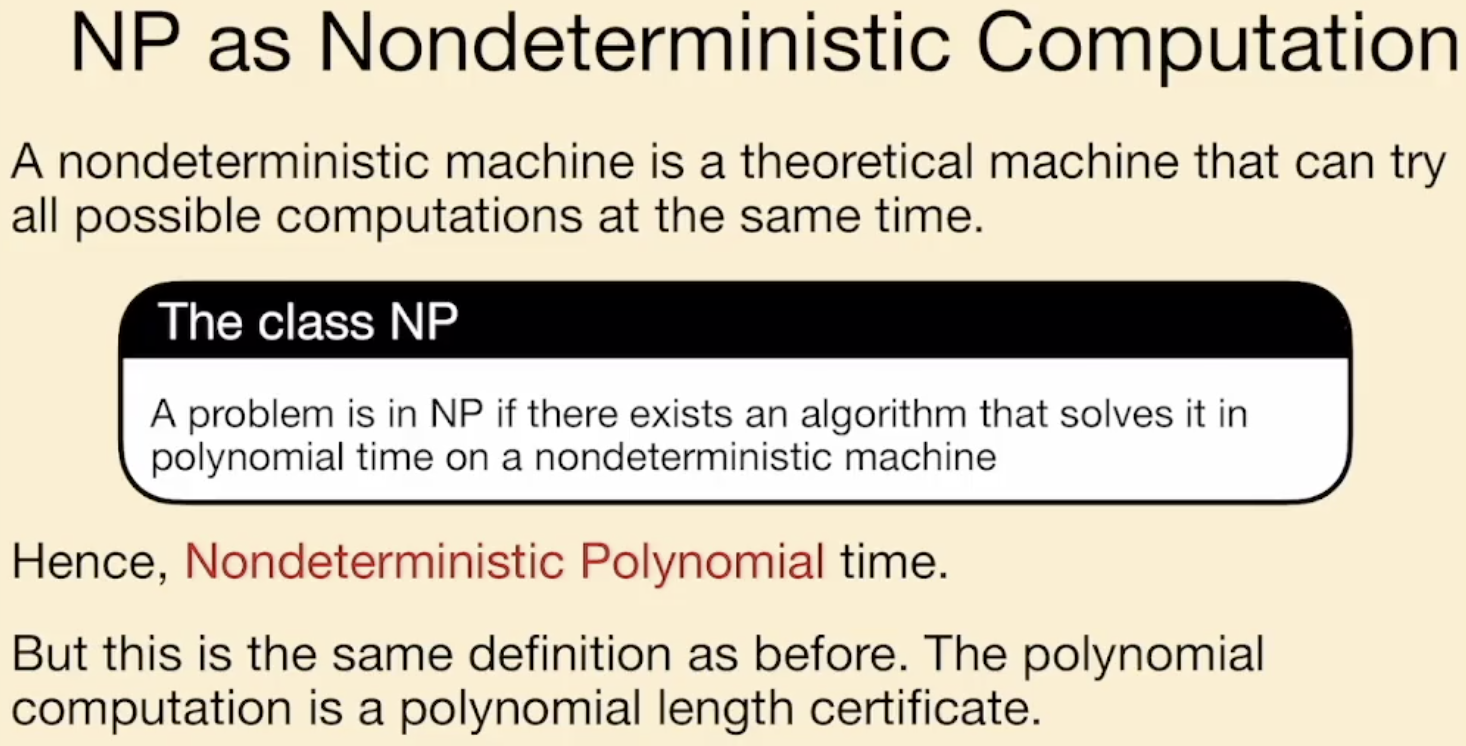### 分析计算困难度时对问题实际困难程度简化的技术 (Reduction)1. $L_1$ 简单不意味着 $L_2$ 一定简单, 但 $L_1$ 难一定意味着 $L_2$ 至少不会更简单.
2. $L_2$ 难不意味着 $L_1$ 一定难, 但 $L_2$ 简单一定意味着 $L_1$ 至少不会更难.
3. 偏序关系 $\leqslant_{P}$ 是具有 传递性 的.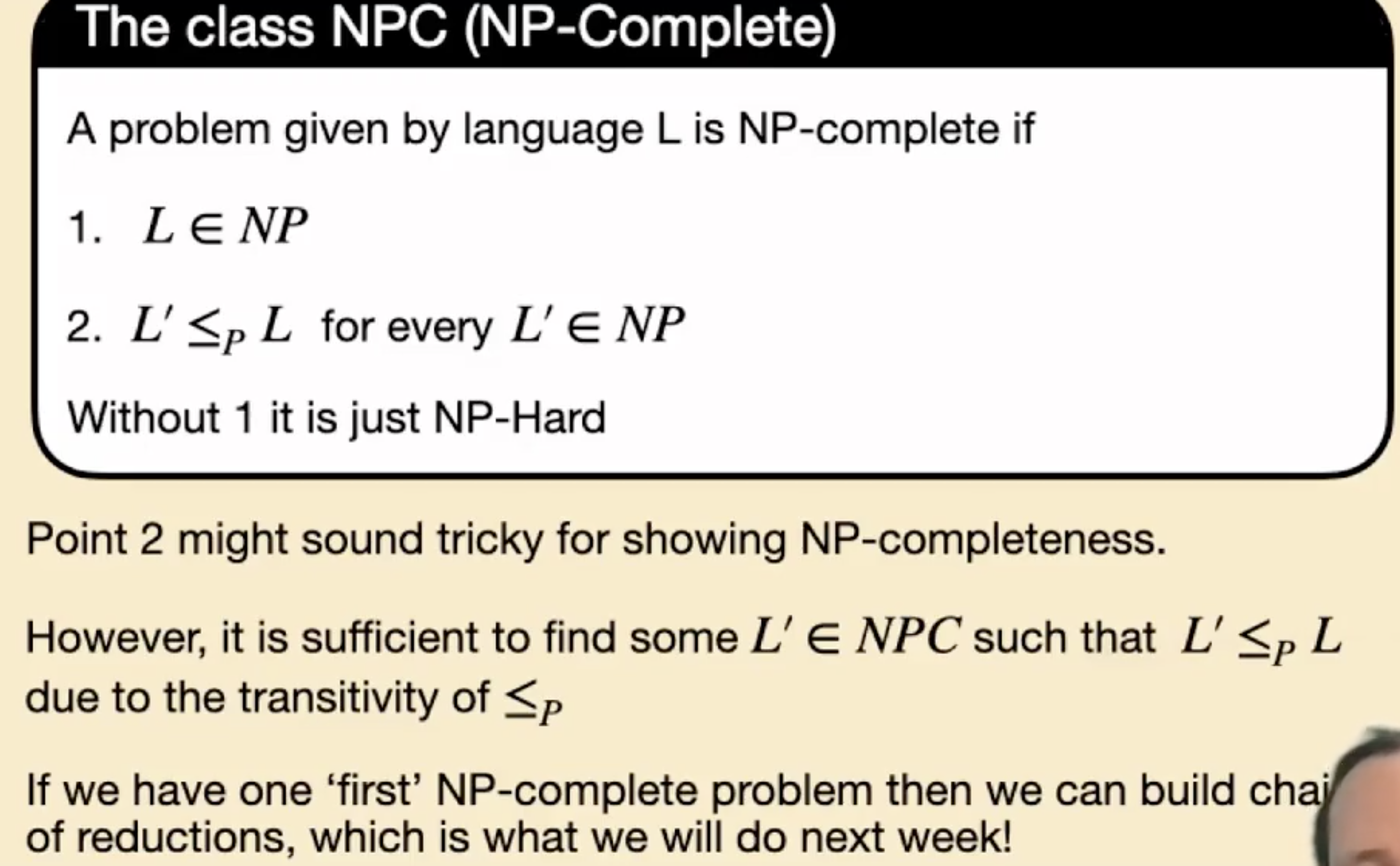1. $L$ 也是 NP 的.
2. $L$ 同时不比 其他任何 NP 问题简单, 也就是说 “任何其他 NP 问题都可 在多项式时间内reduceL”.

## 大脑升级: 重要 NP-Complete 问题一览

### SAT 问题系列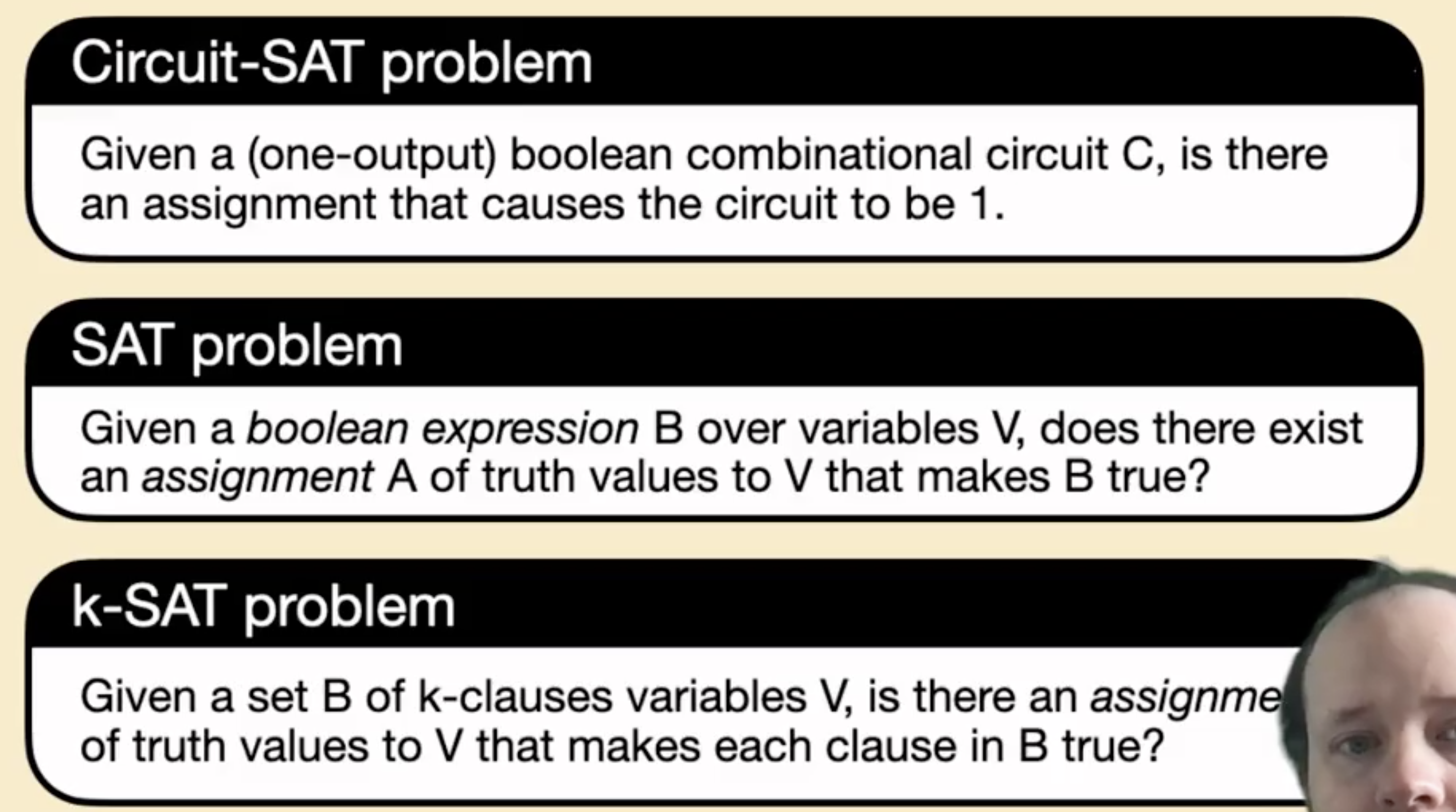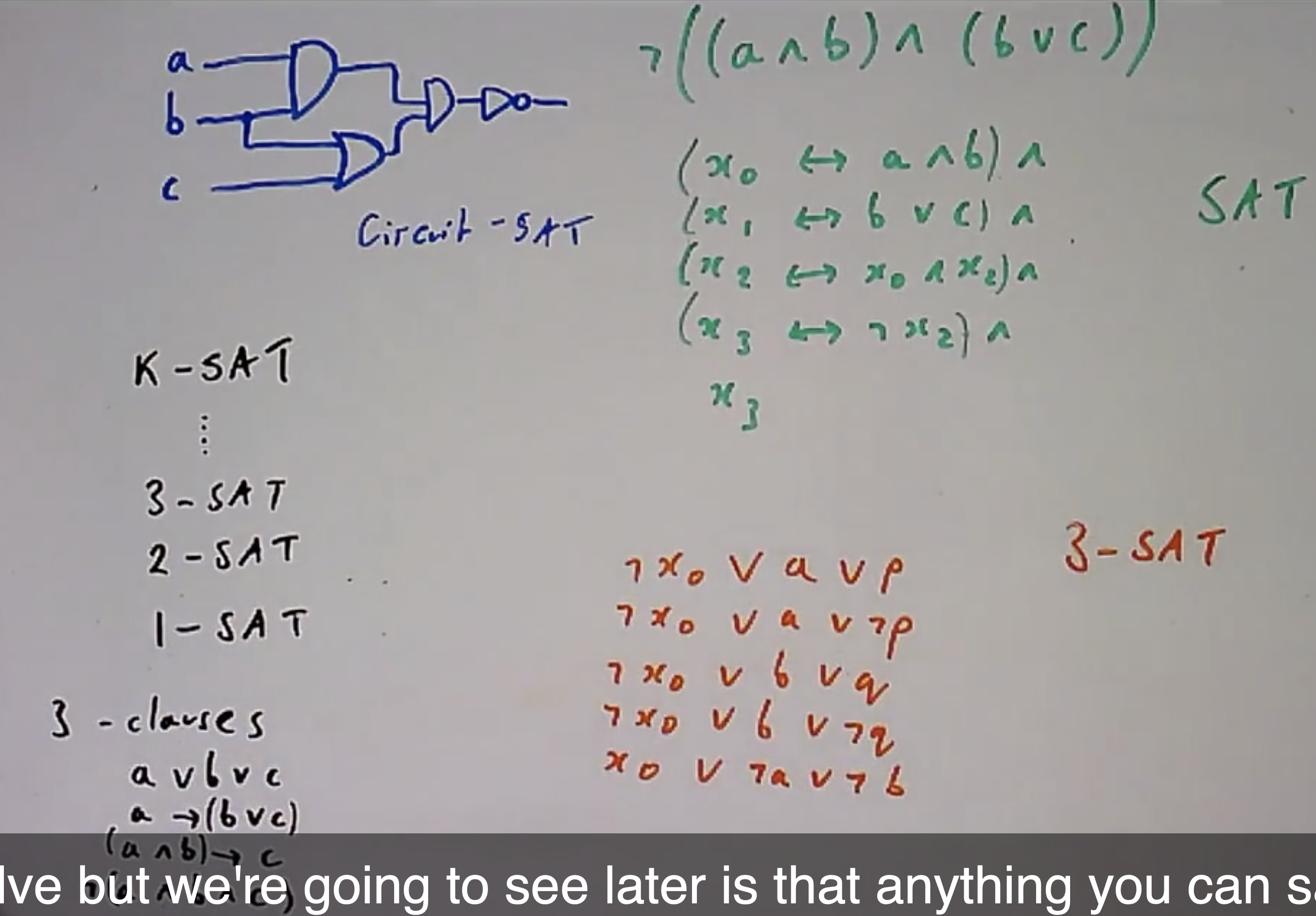### 图相关问题系列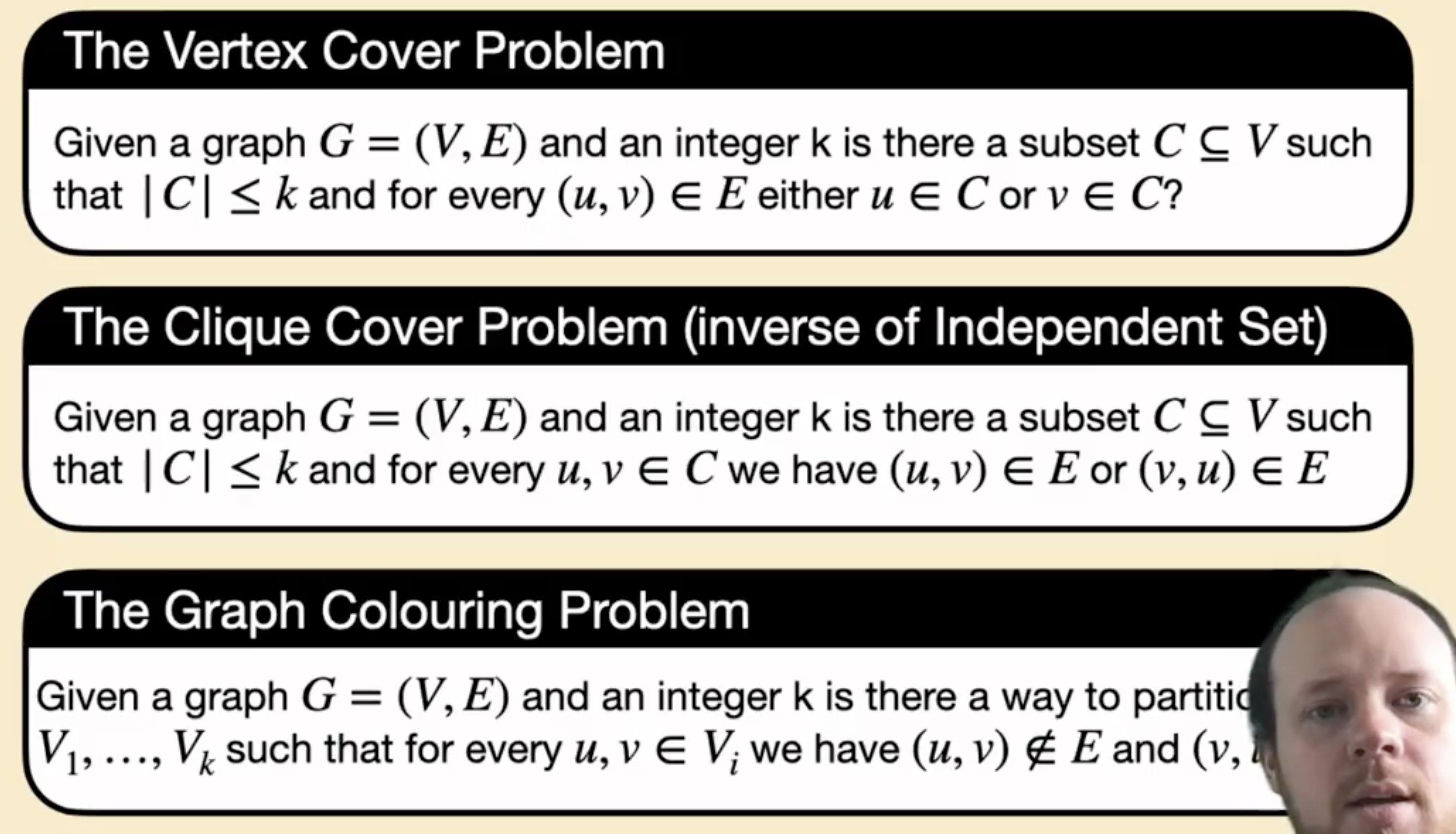1. Vertex Cover Problem: 寻找顶点集 $V$ 的子集 $C$ 使图中每一条边中的至少一个顶点都在这个子集中.
2. Clique Cover Problem: 寻找大小不超过 $k$ 的 连通子图 的顶点集, 其实就是在图中寻找 大小不少于 $\vert V-k\vert$ 的独立集 问题的镜像问题.
3. Graph Coloring Problem: 图染色, 确保任一对相联的顶点染上的颜色不同.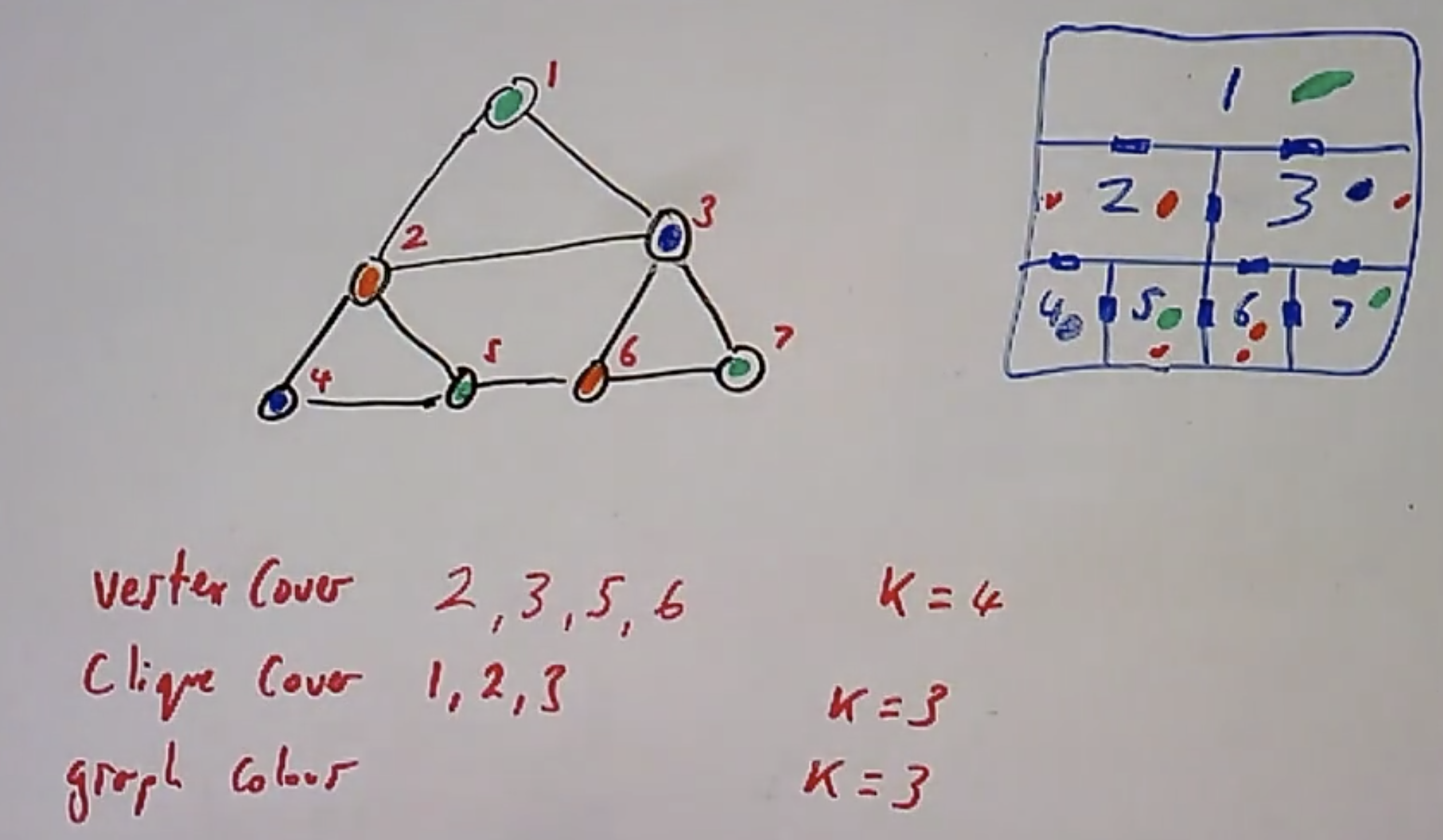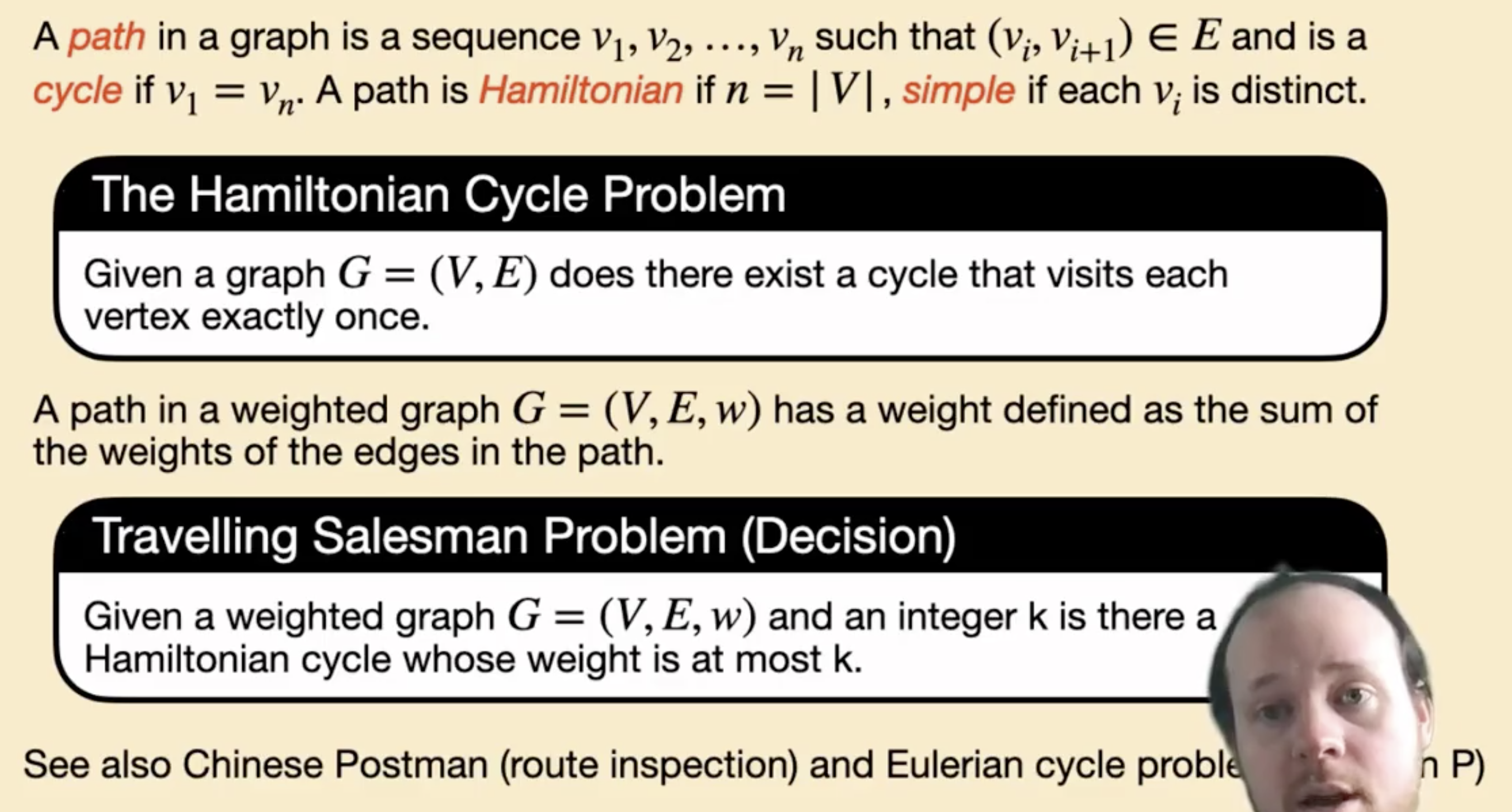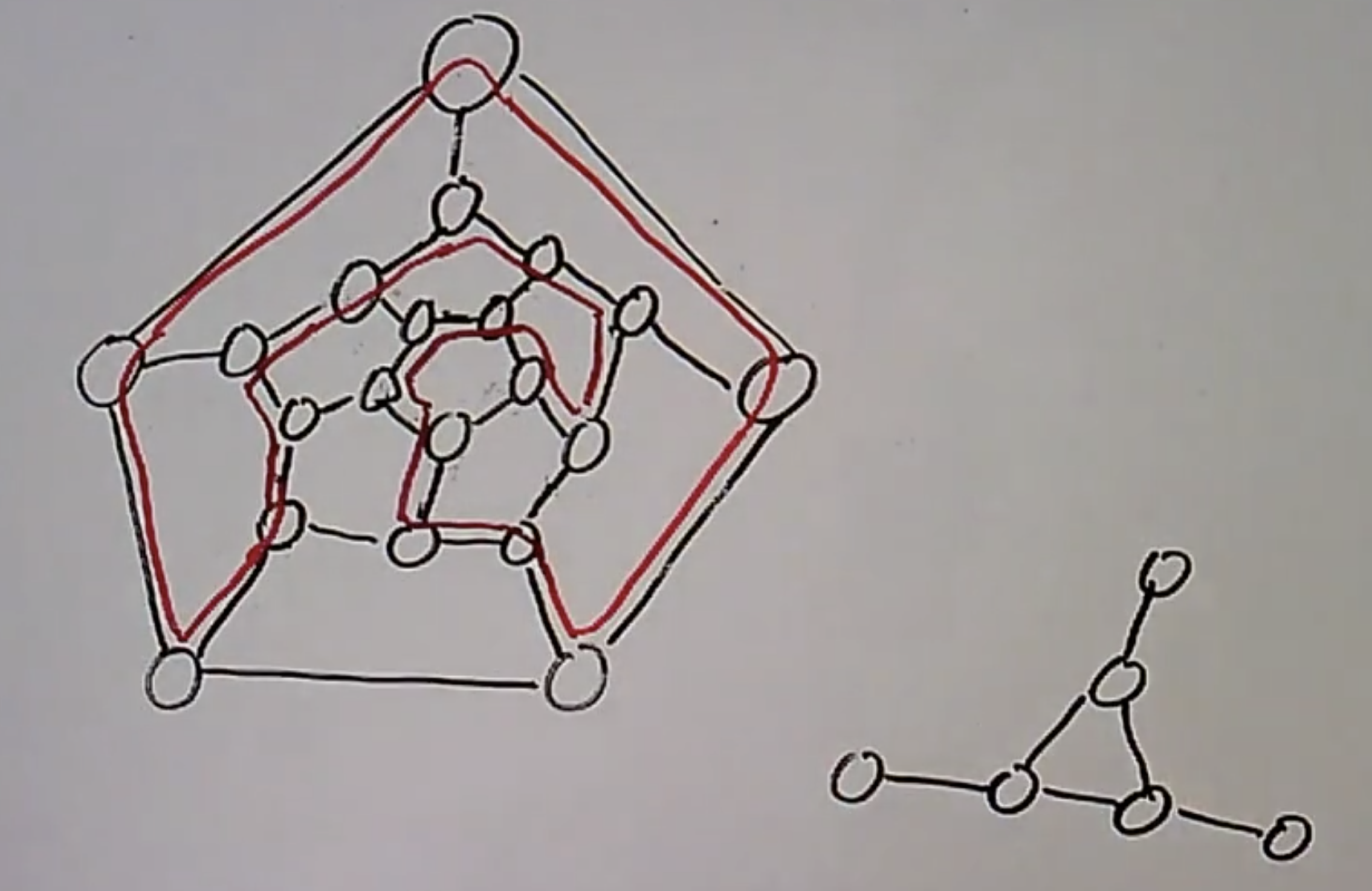### 图中最长/最短路径计算问题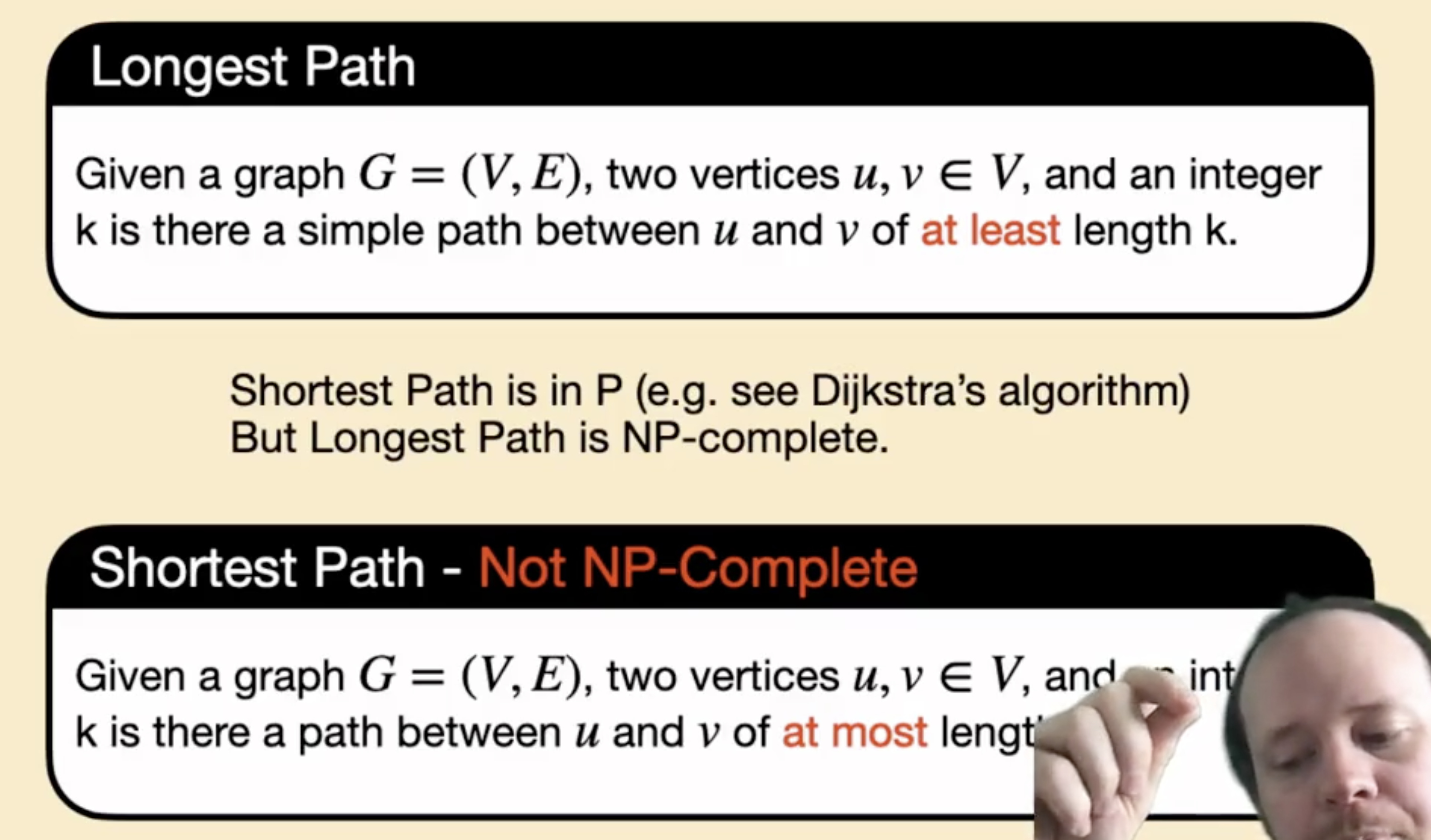### 背包系列问题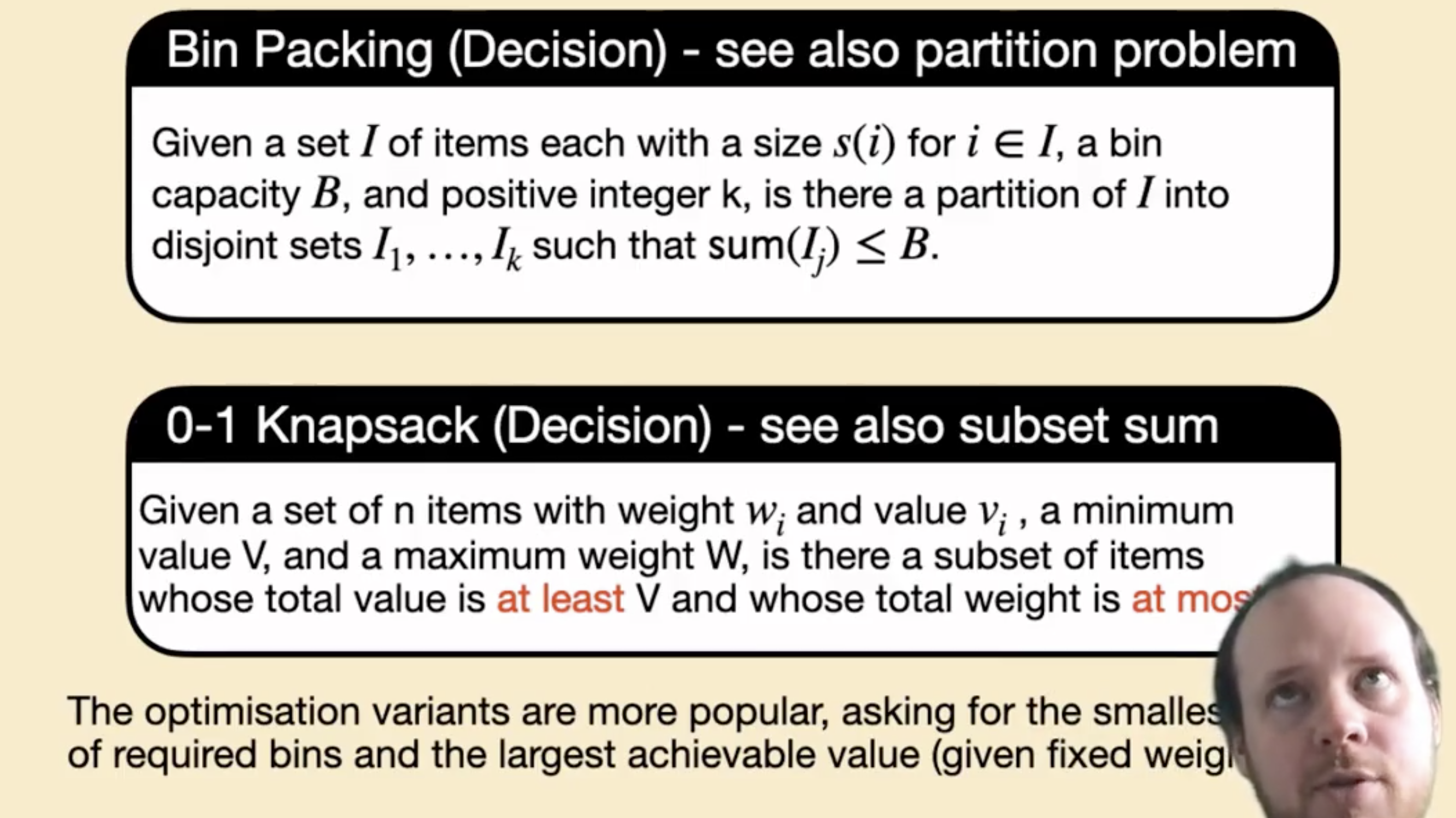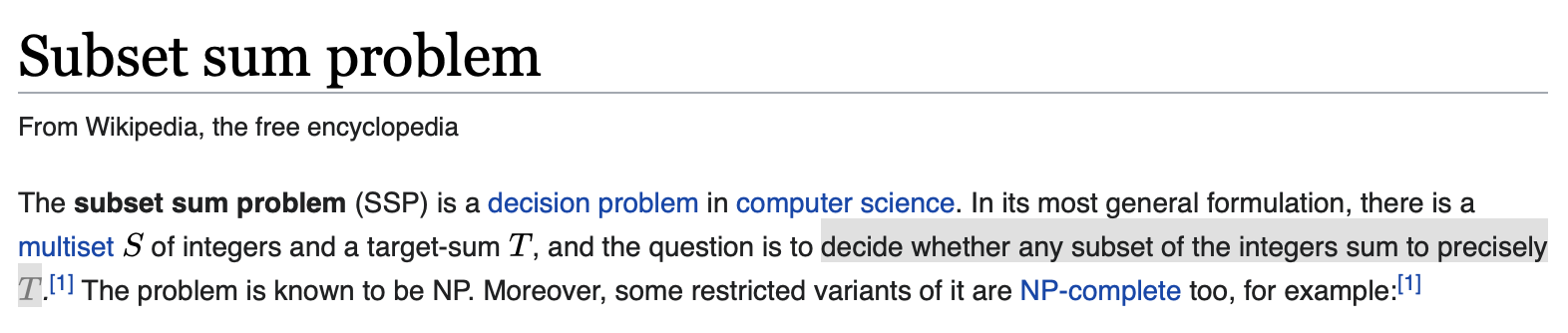### 线性规划问题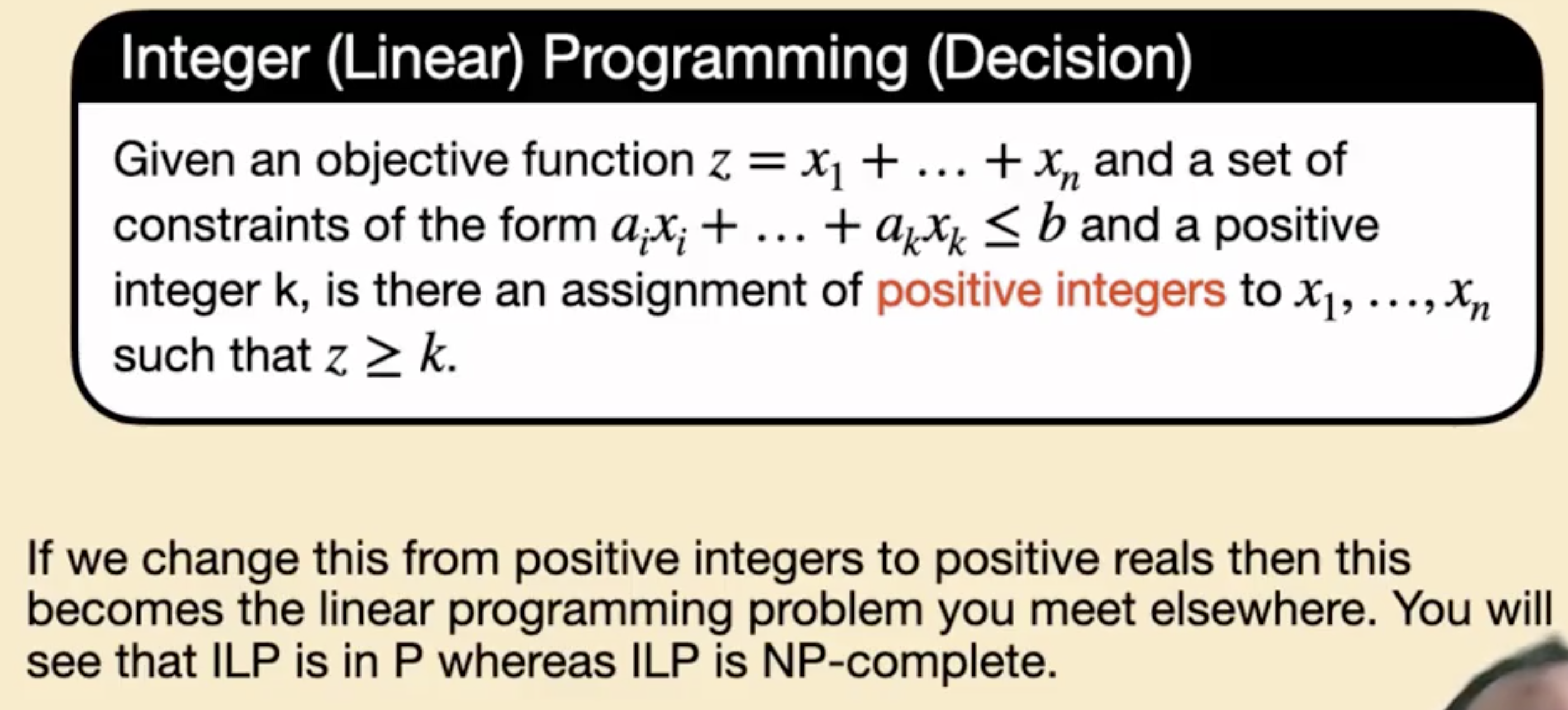## 对可满足性问题的简化 (Reduction)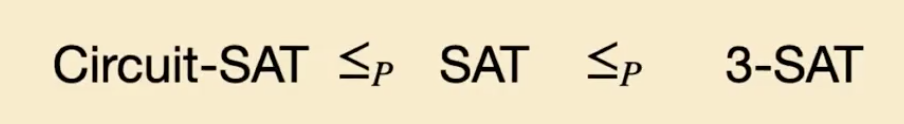### 从任何 NP 问题向 Circuit-SAT 的简化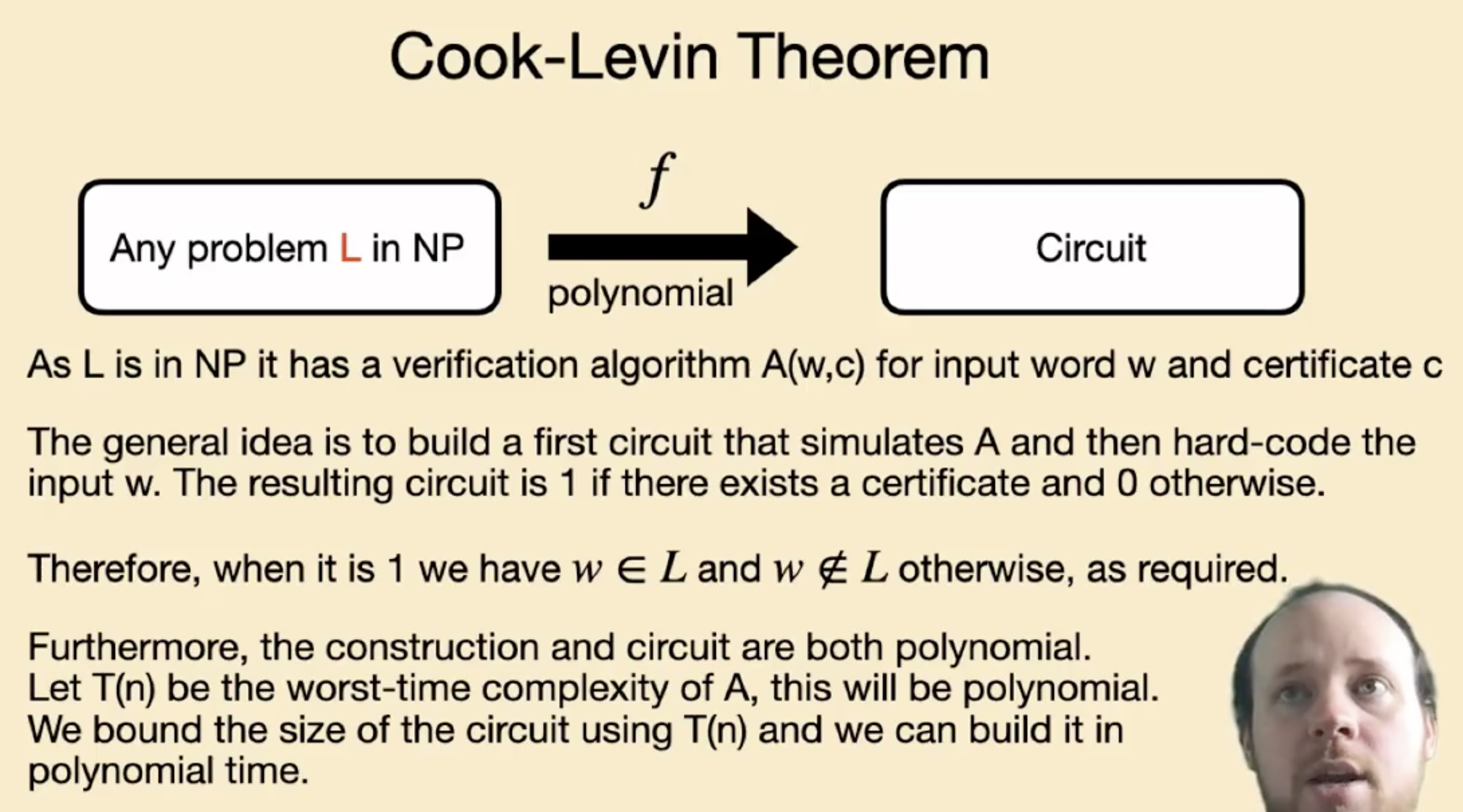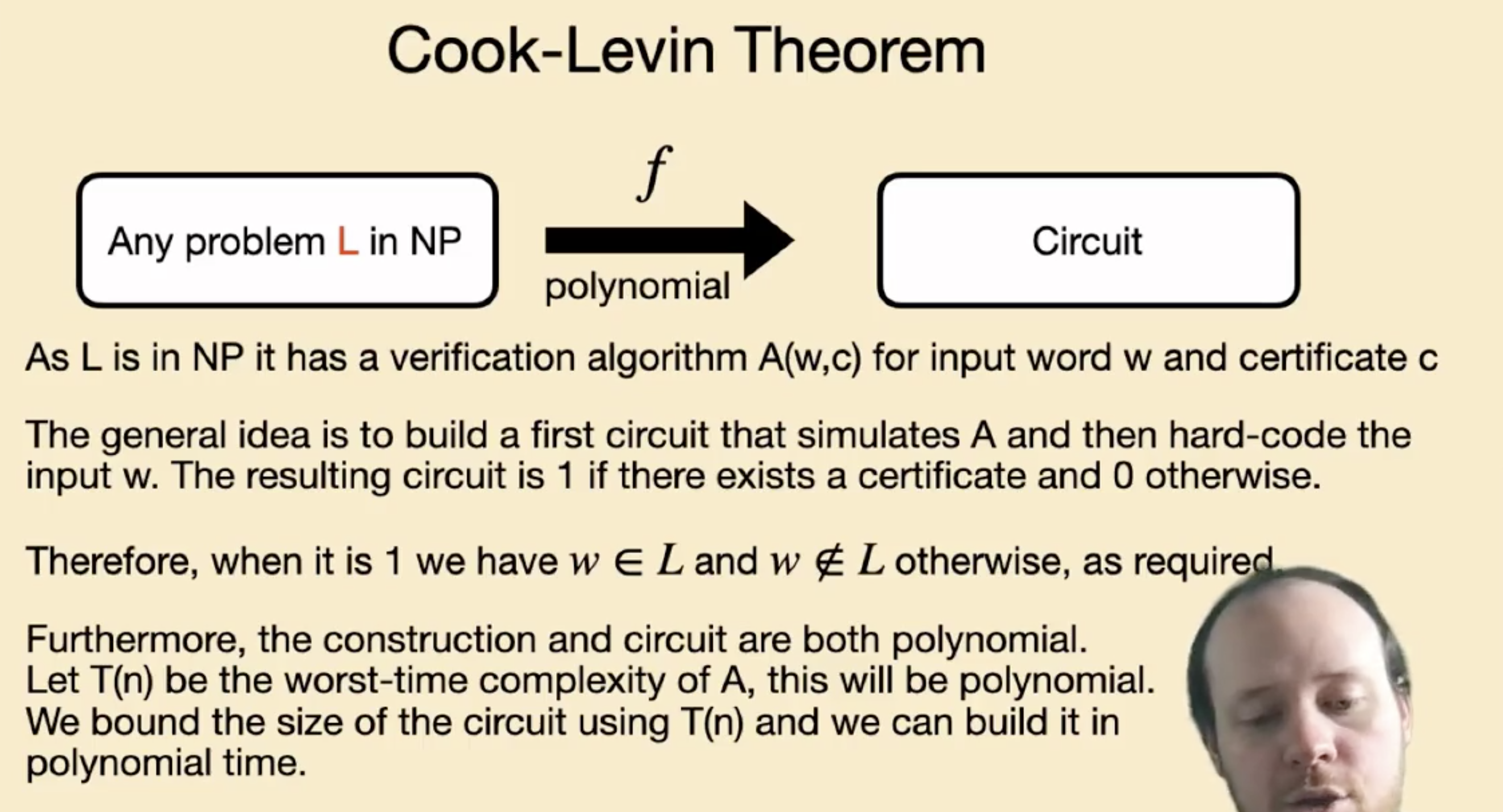(丧心病狂, 在iOS 短信应用存储的 gif 图里用逻辑门搭计算机的恶意软件 Pegasus 的开发者直呼内行….)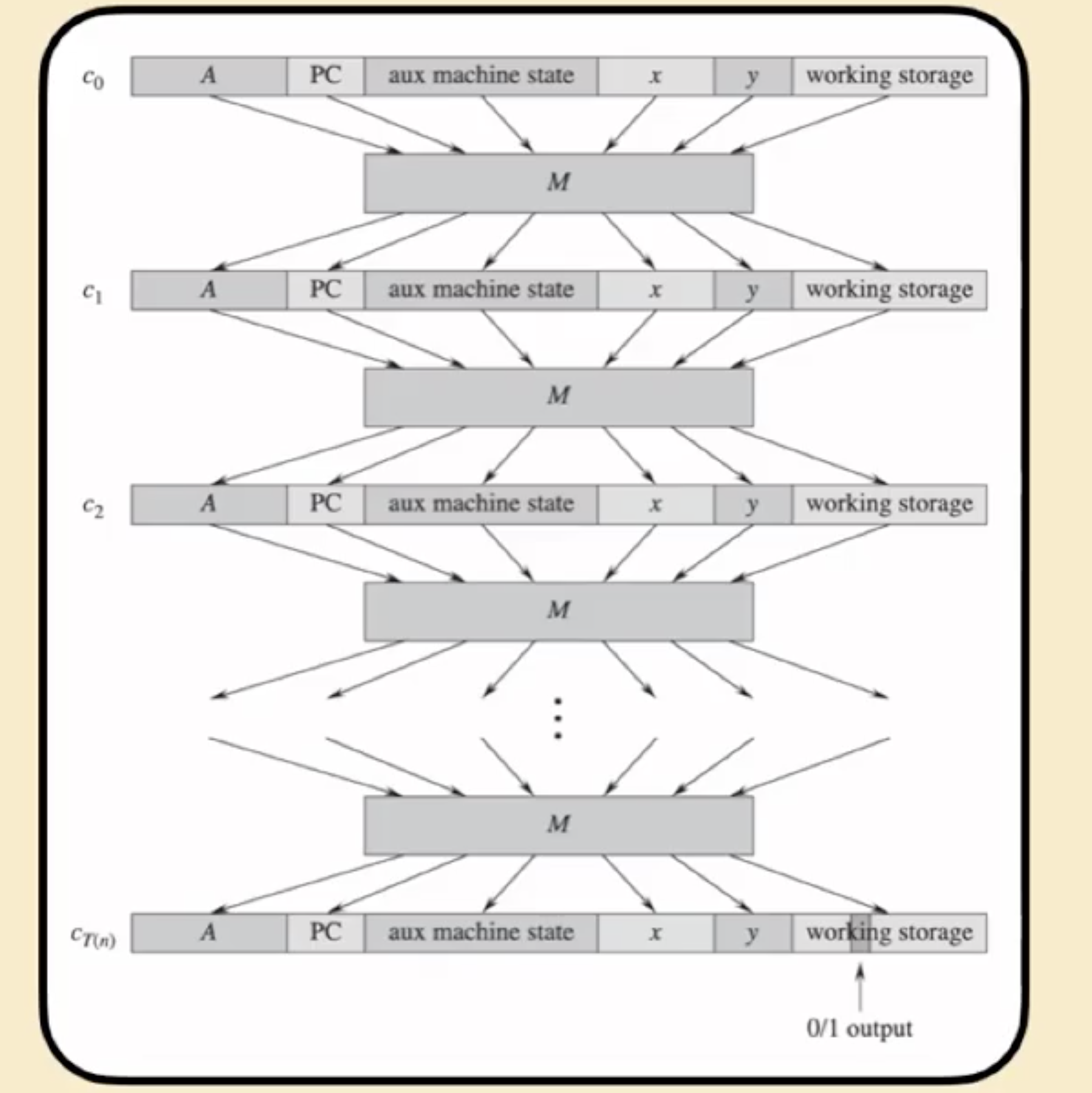### 从 Circuit-SAT 向 SAT 的简化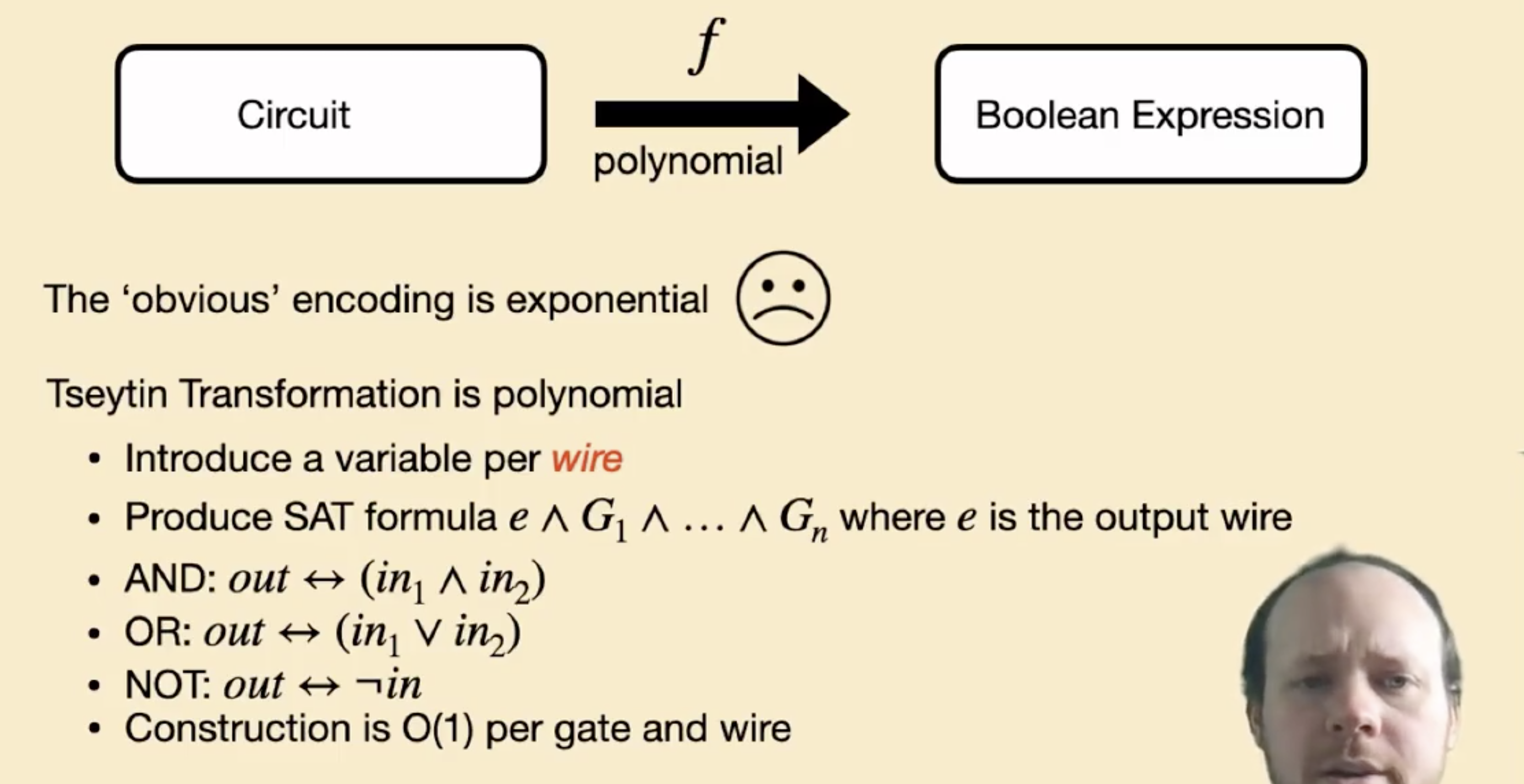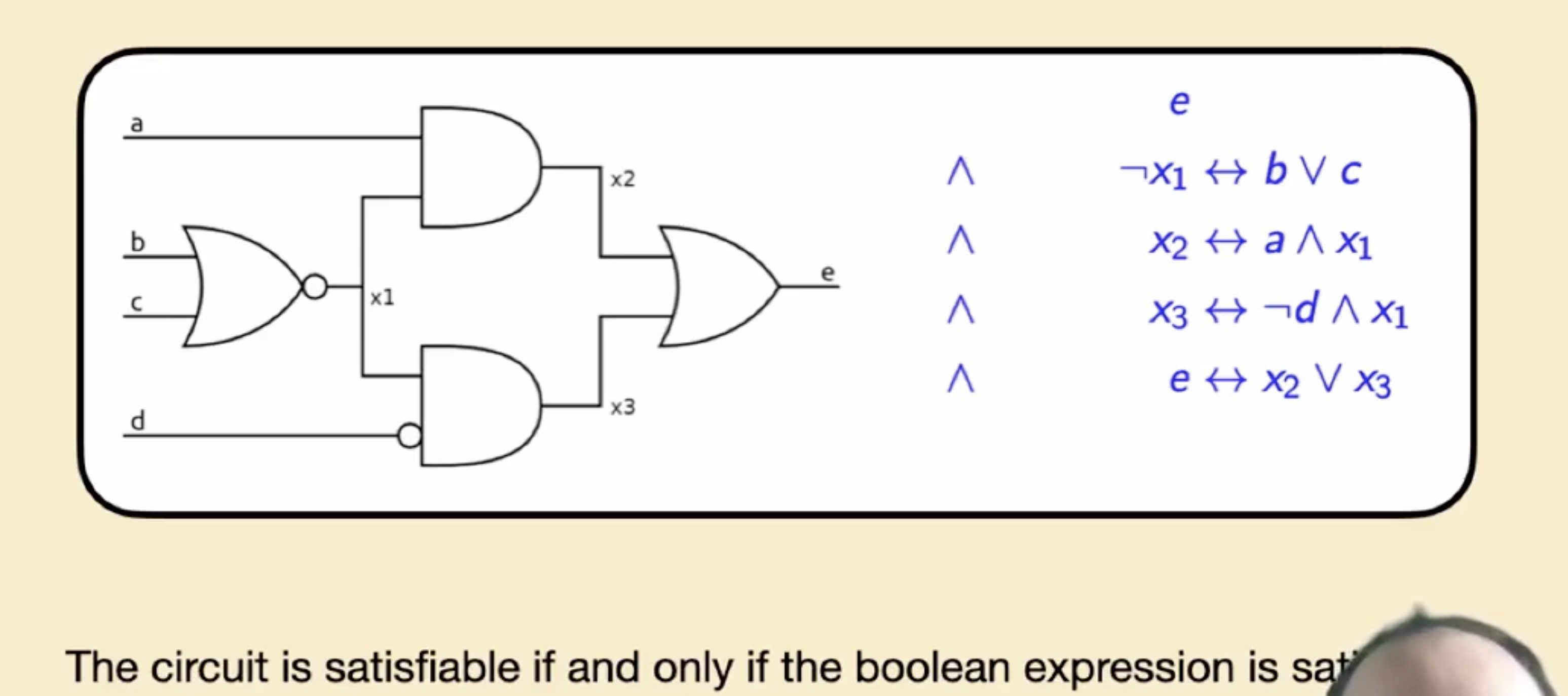### 从 SAT 向 3-SAT 的简化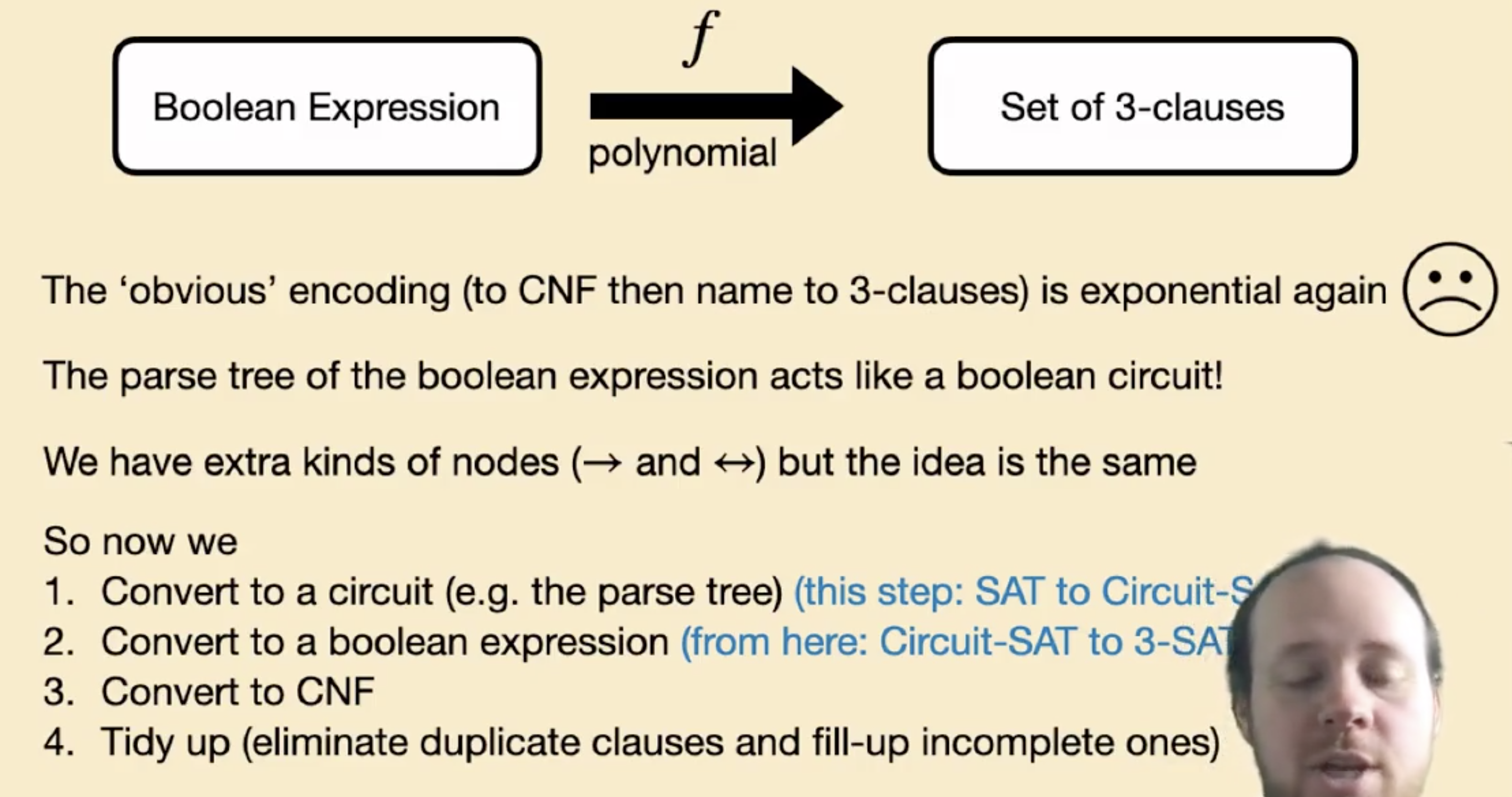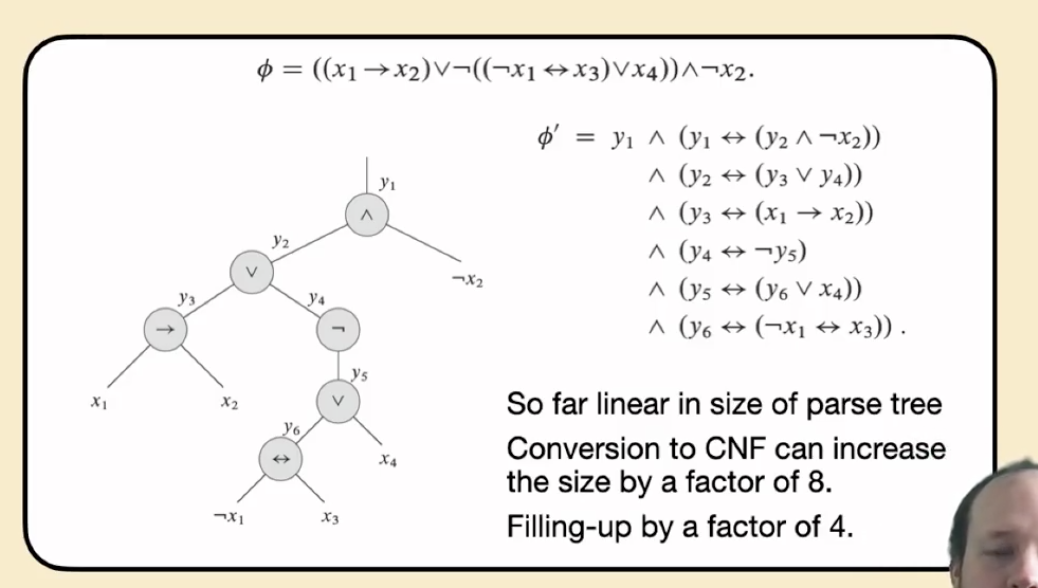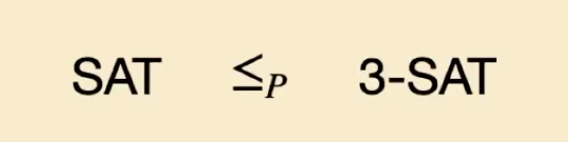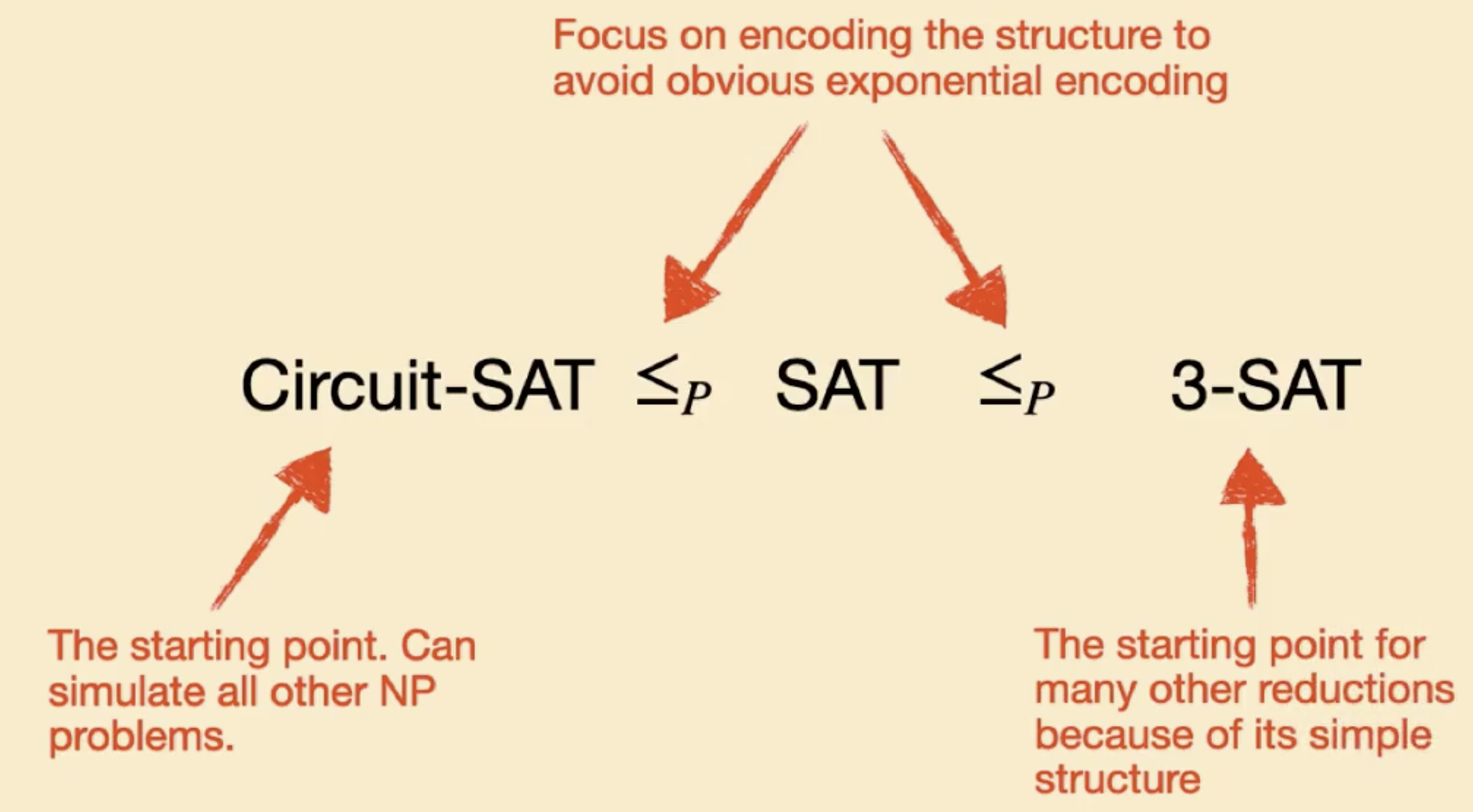### 从 3-SAT 向 Clique 的简化

Clique 问题实际上就是考察: 给定图 $G$ 和约束 $k$, 问该图中是否存在大小为 $k$ 的连通子图.

1. 在同一个三元组中的顶点 永不相连.
2. 不连接 符号相同正负性质不同 的顶点, 如形如 $a_1$ 和 $\neg a_1$ 的顶点就不能相互连接, 但 $a_1$ 和 $\neg b_2$ 就可相连.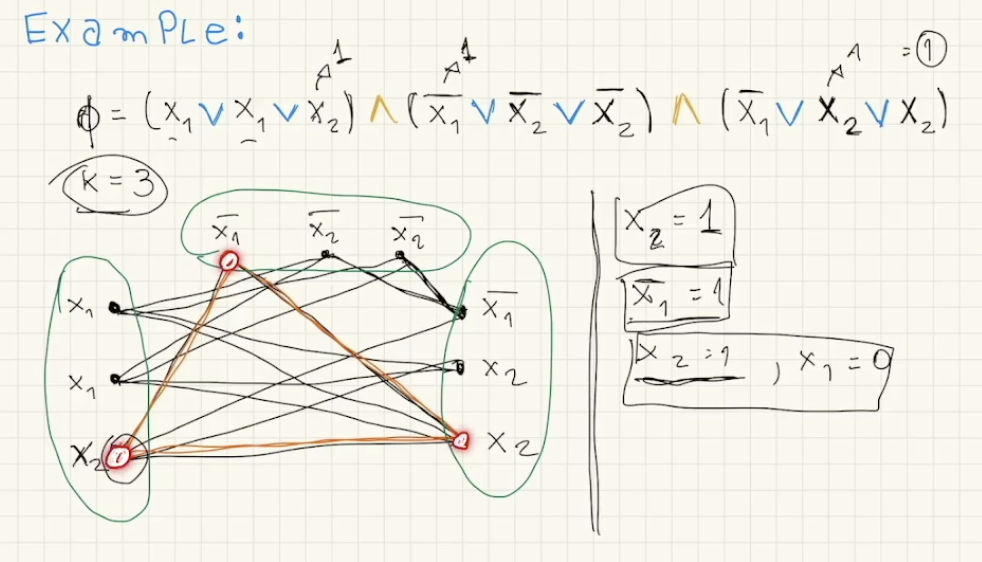### 对图相关问题的简化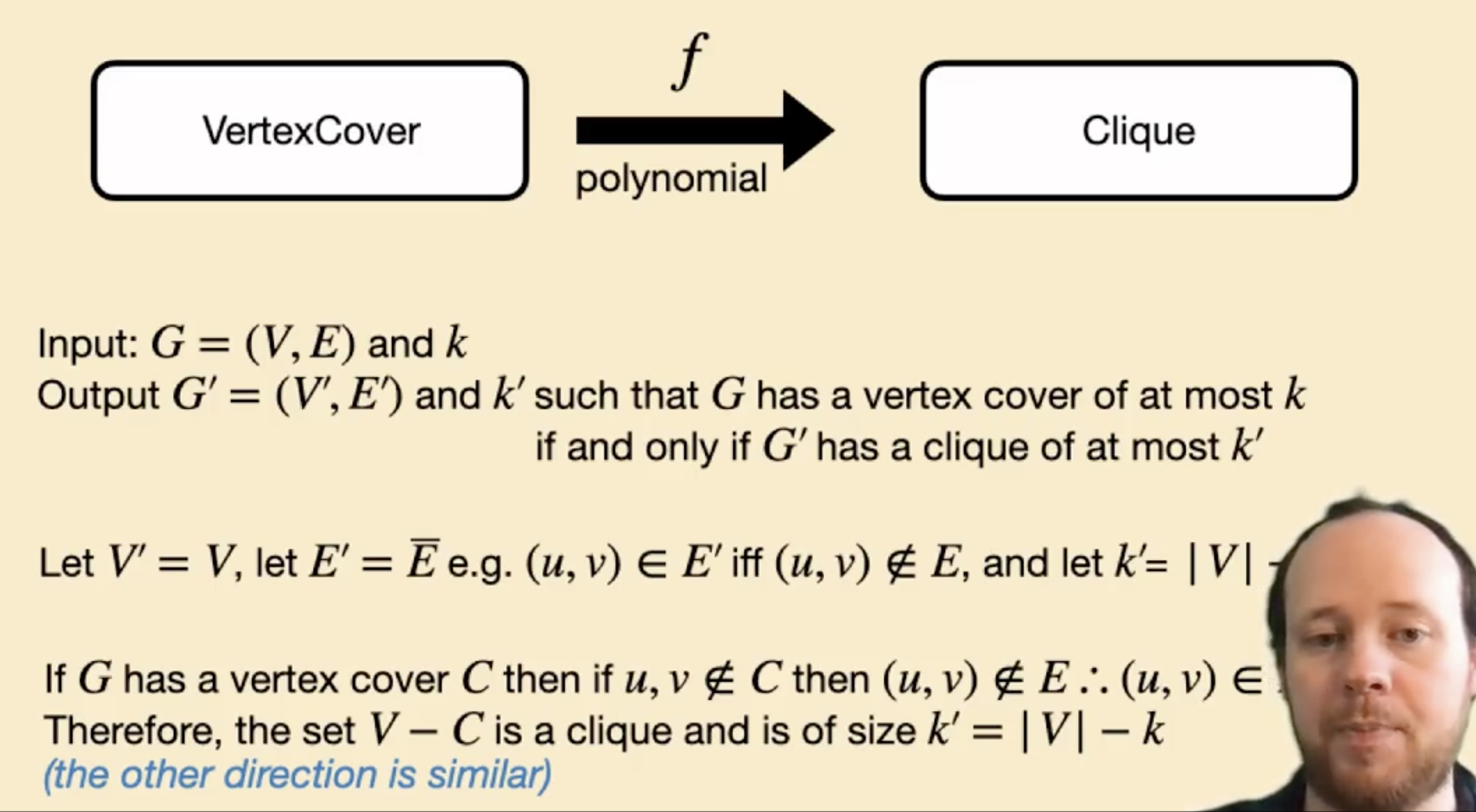1. $V’ = V$
2. $E’ = \bar{E}$, 也就是对 $E$ 在 全体可能边集 中的 取反.
3. $k’ = \vert V \vert -k$.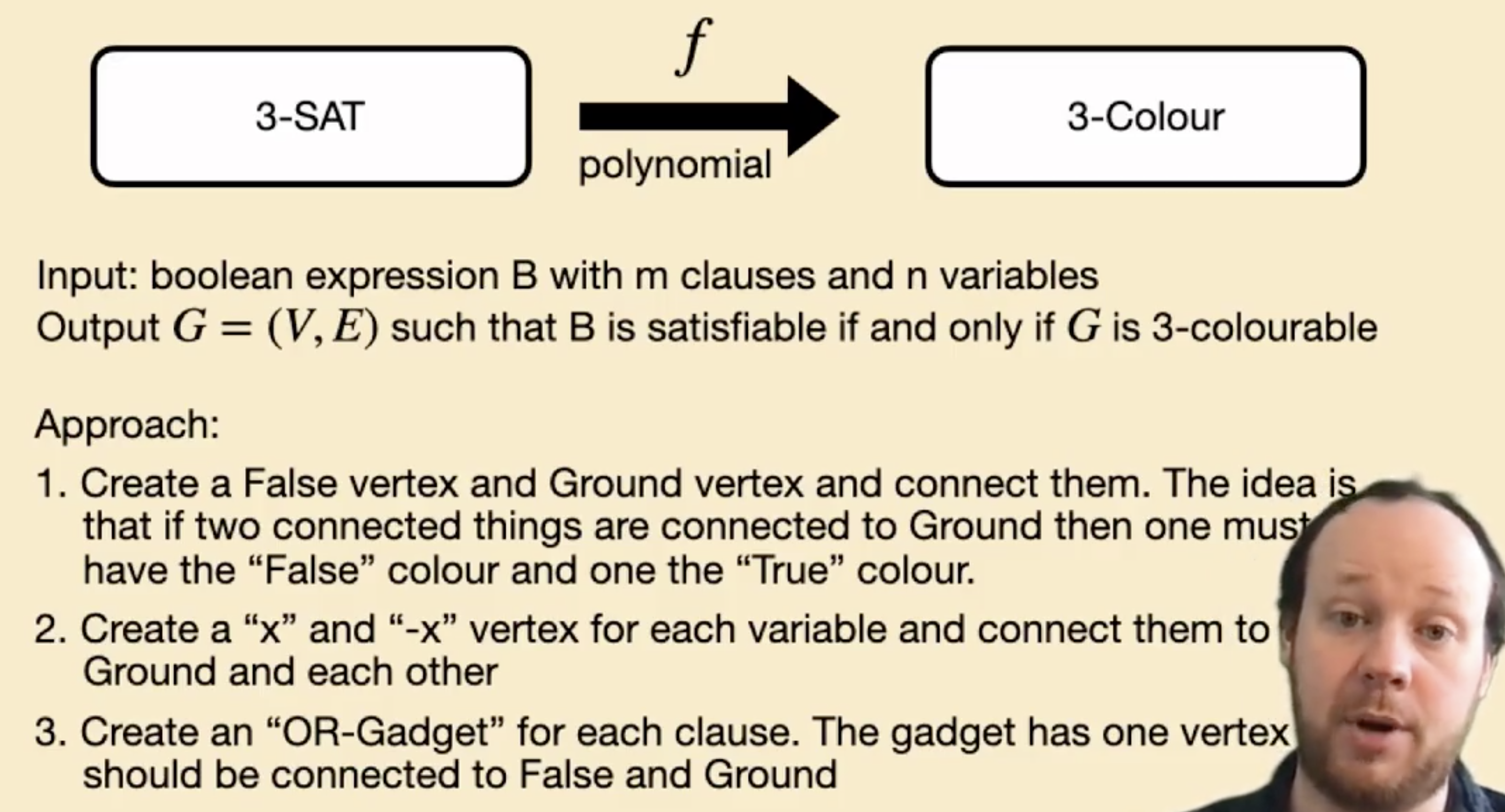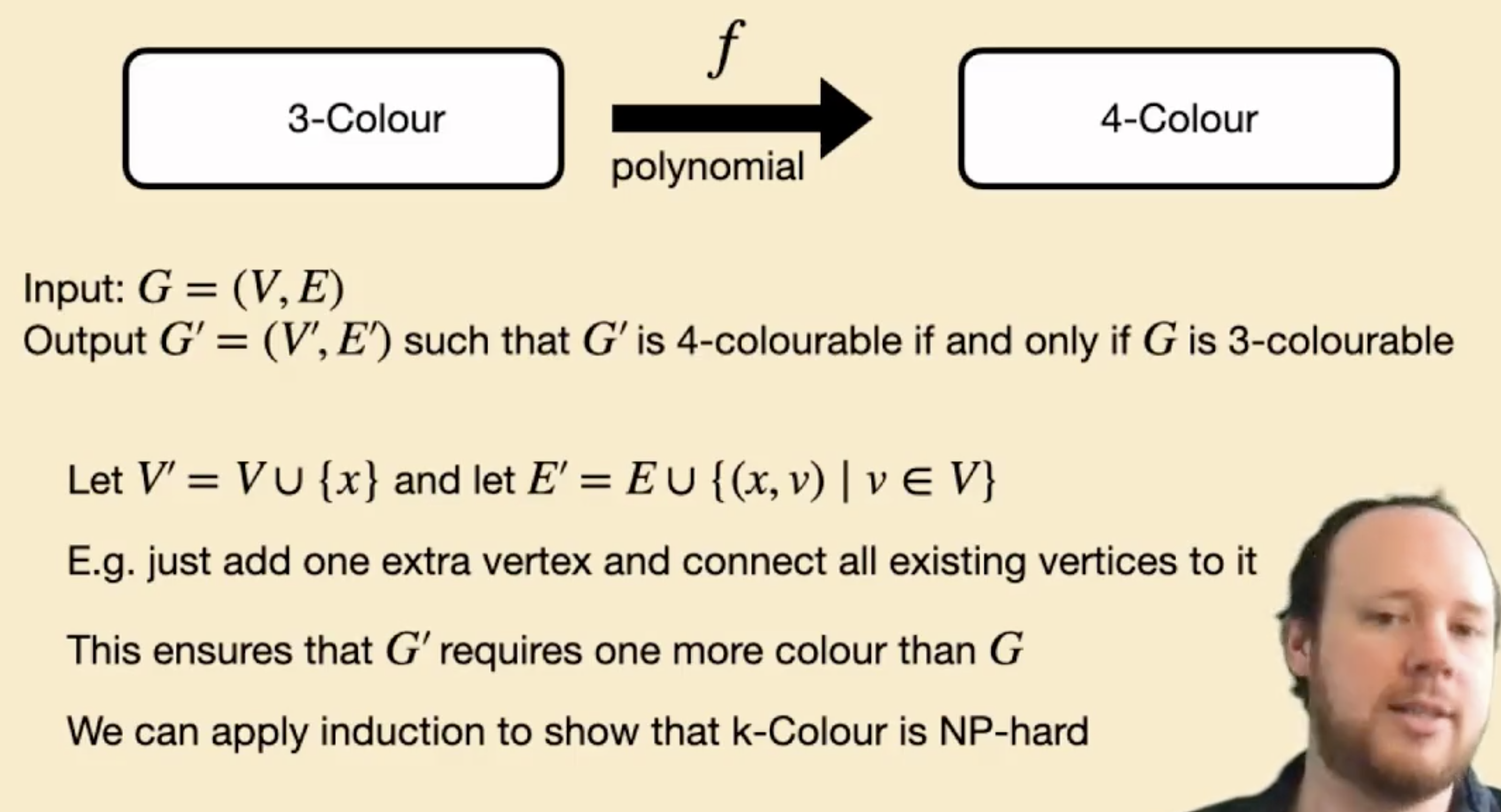(这里有时间再补, 没时间就算了, 记结论就行)

1. Circuit-SAT
2. SAT
3. 3-SAT/k-SAT with k >= 3
4. Vertex Cover: find a subset C of V so that for each edge in E at least one node is in C given the constraint on maximum size of C
5. Clique: inverse question on independent sets, find complete sub graph’s vertex set C with constraint on the maximum size of C.
6. Graph Colorng: assign color to every nodes, but each pair of neighbors cannot share the same color.
7. Hamiltonian Cycle: find a shortest circle in graph that connect every vertex.
8. Bin Packing: separate the set into k subsets where each subset’s total weight is no more than P.
9. 0-1 knapsack: given a list of weighted items and a bag no heavier than P, find an optimal combination of items to pack into the bag so that the weight constraint does not exceed but the total value can be maximised.
10. Integer programming: stricter linear programming, where each variables’ value can only be integer.

### 相关习题解析